# ML Aggarwal Solutions for Class 10 Maths Chapter 6 Factorization

ML Aggarwal Solutions for Class 10 Maths Chapter 6 Factorization are provided here. Our expert faculty team has prepared solutions to help students in their exam preparation to obtain good marks in Maths. If you wish to secure an excellent score, solving ML Aggarwal Solutions is an utmost necessity. Scoring high marks requires a good amount of practice on each and every topic. These solutions will help you in gaining knowledge and a strong command over the subject. Practising the textbook questions will help you in analyzing your level of preparation and knowledge of the concept.

Chapter 6 – Factorization solutions are available for download in pdf format and provide solutions to all the questions provided in ML Aggarwal Solutions for Class 10 Maths Chapter 6. Factorization is when you break a number down into smaller numbers that, multiplied together, give you that original number. When you split a number into its factors or divisors, it is called factorization. Now, let us have a look at some of the important concepts discussed in this chapter.

• Polynomial and Related terms
• Polynomial Equations
• Equality of Two Polynomials
• Division Algorithm for Polynomials
• Factors of Polynomial
• Root of a Polynomial Equations
• Remainder Theorem
• Factor Theorem
• Use of Factor Theorem

## ML Aggarwal Solutions for Class 10 Maths Chapter 6 :-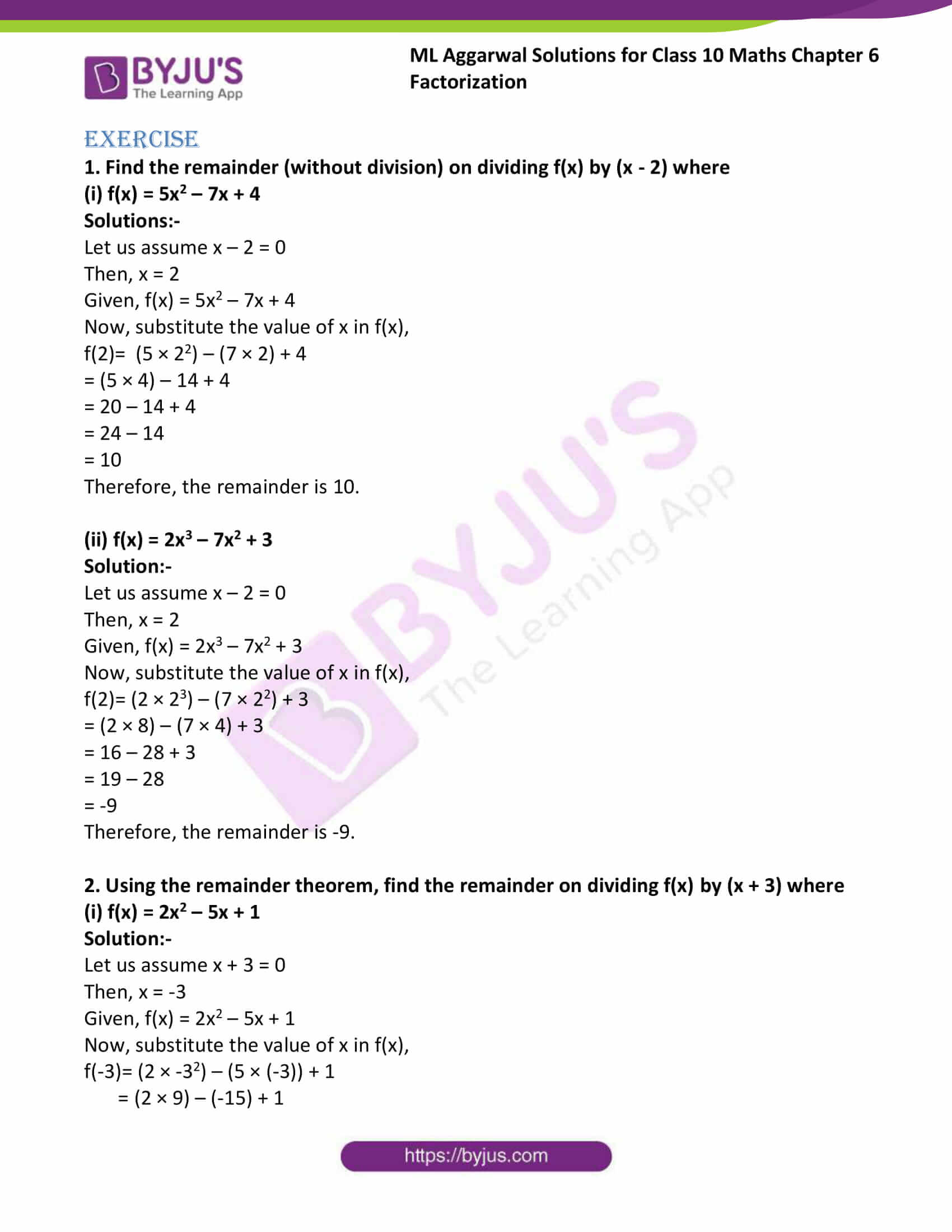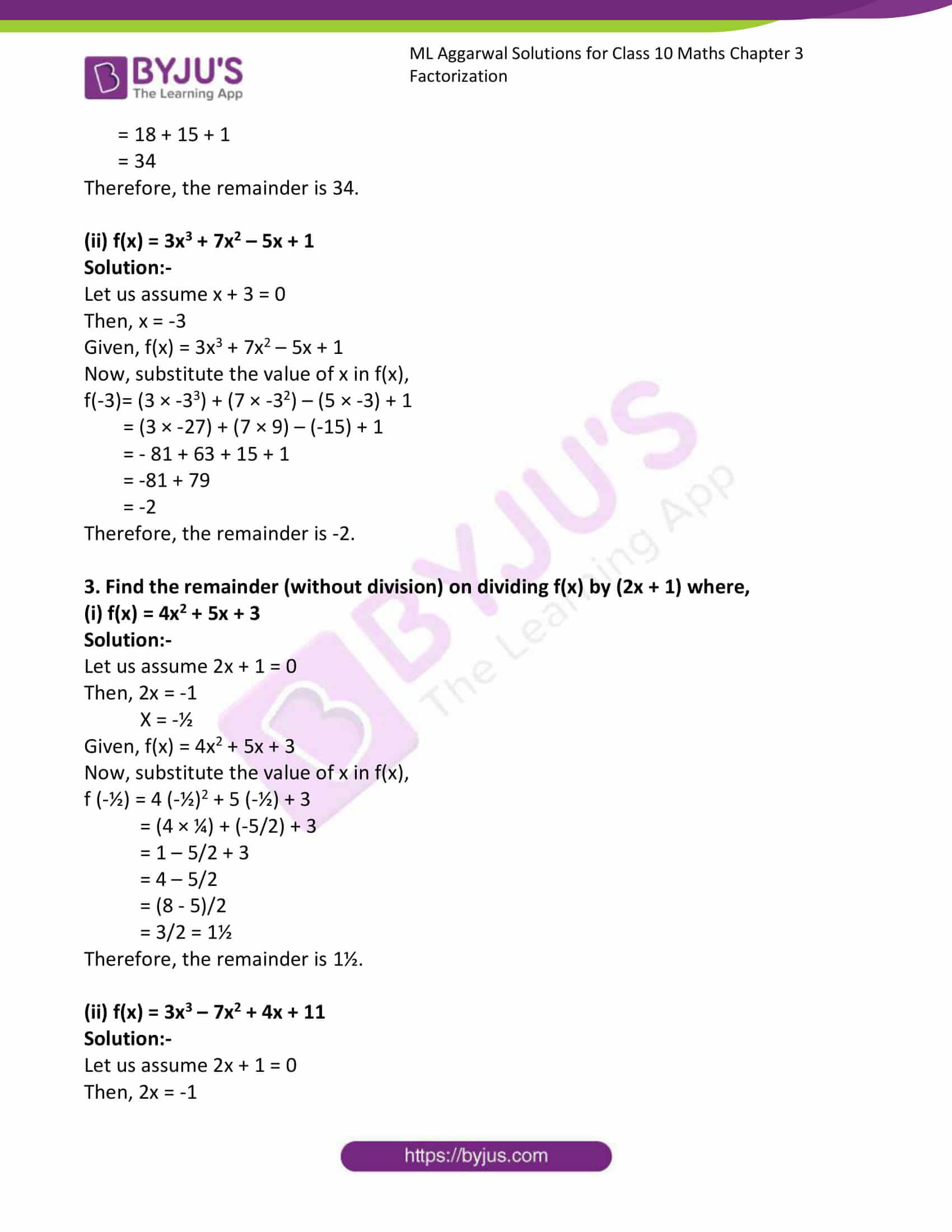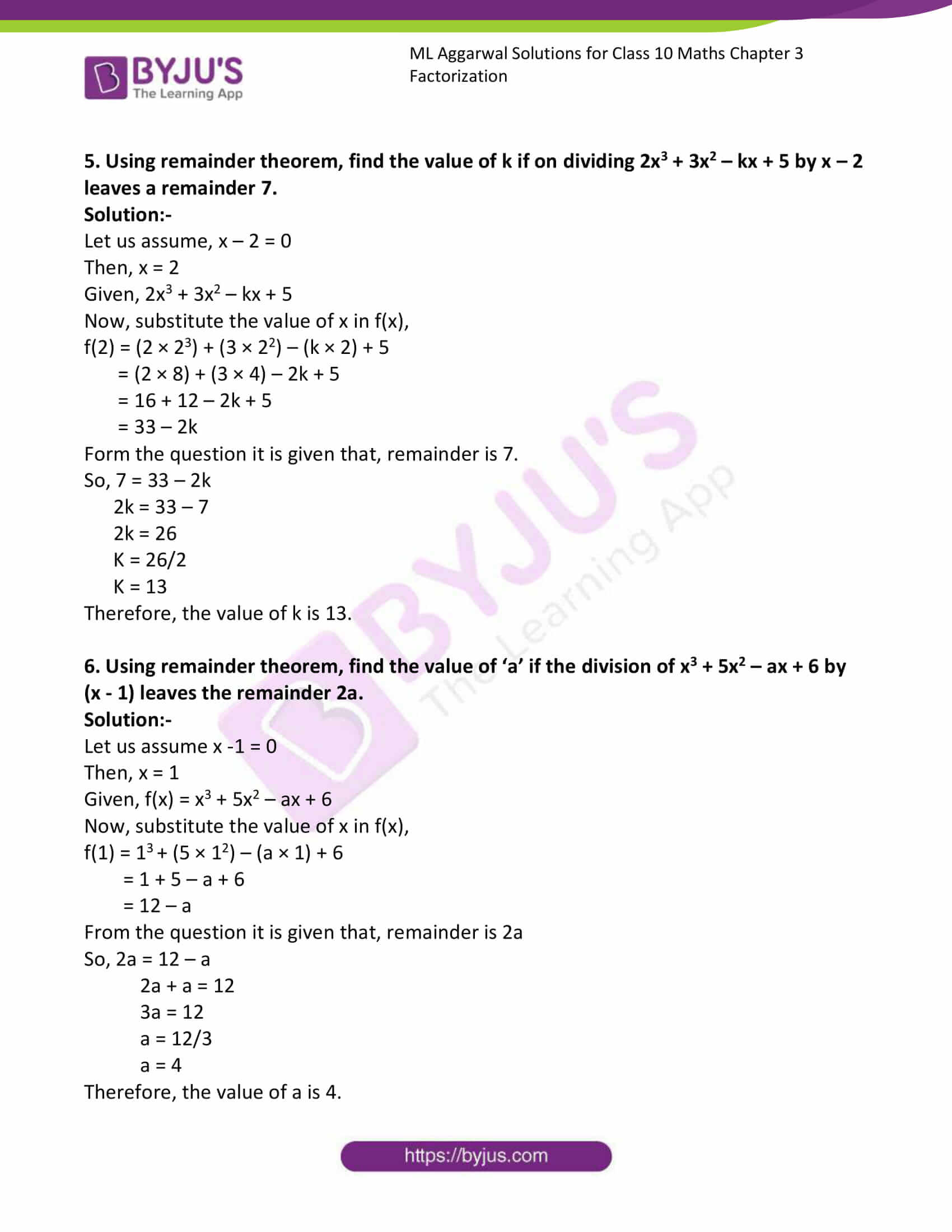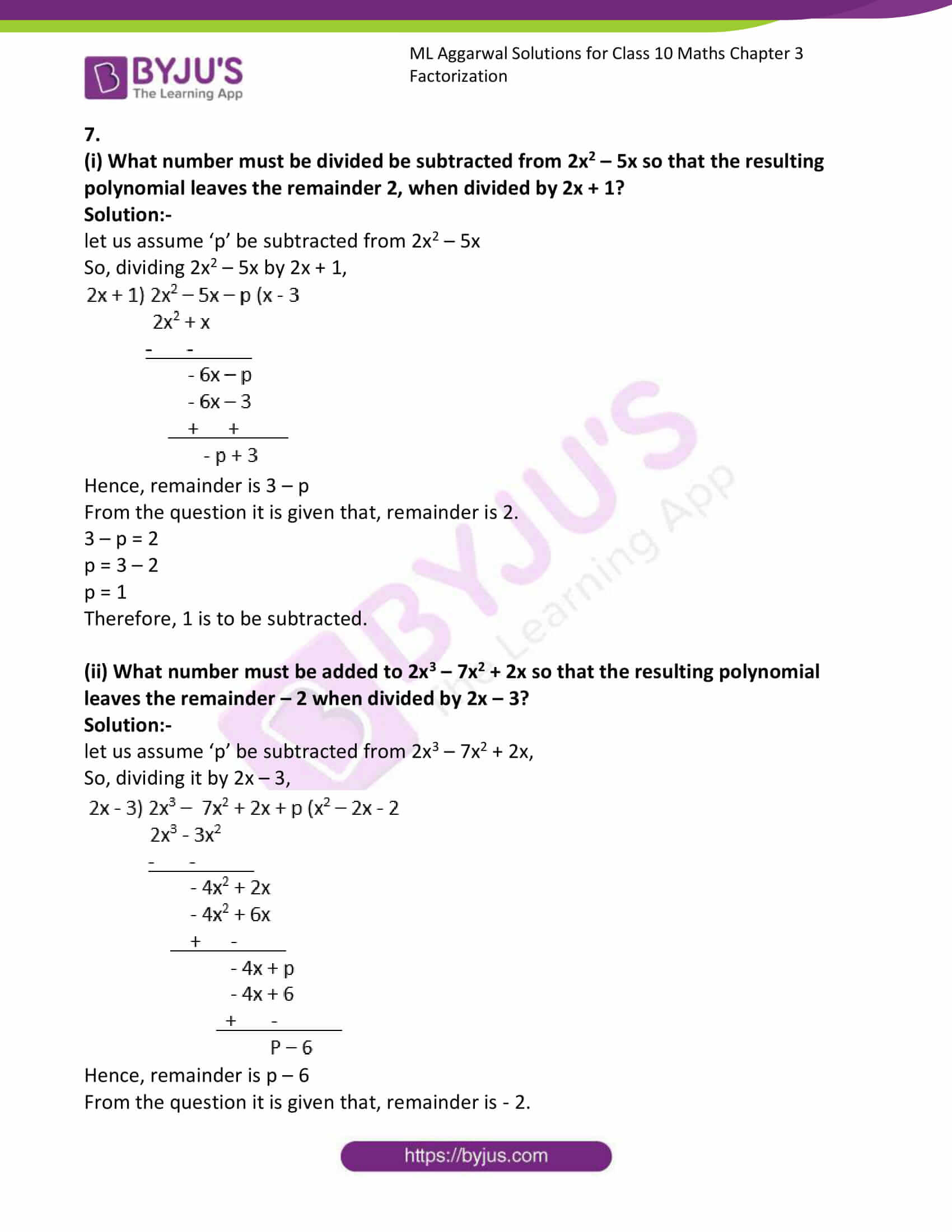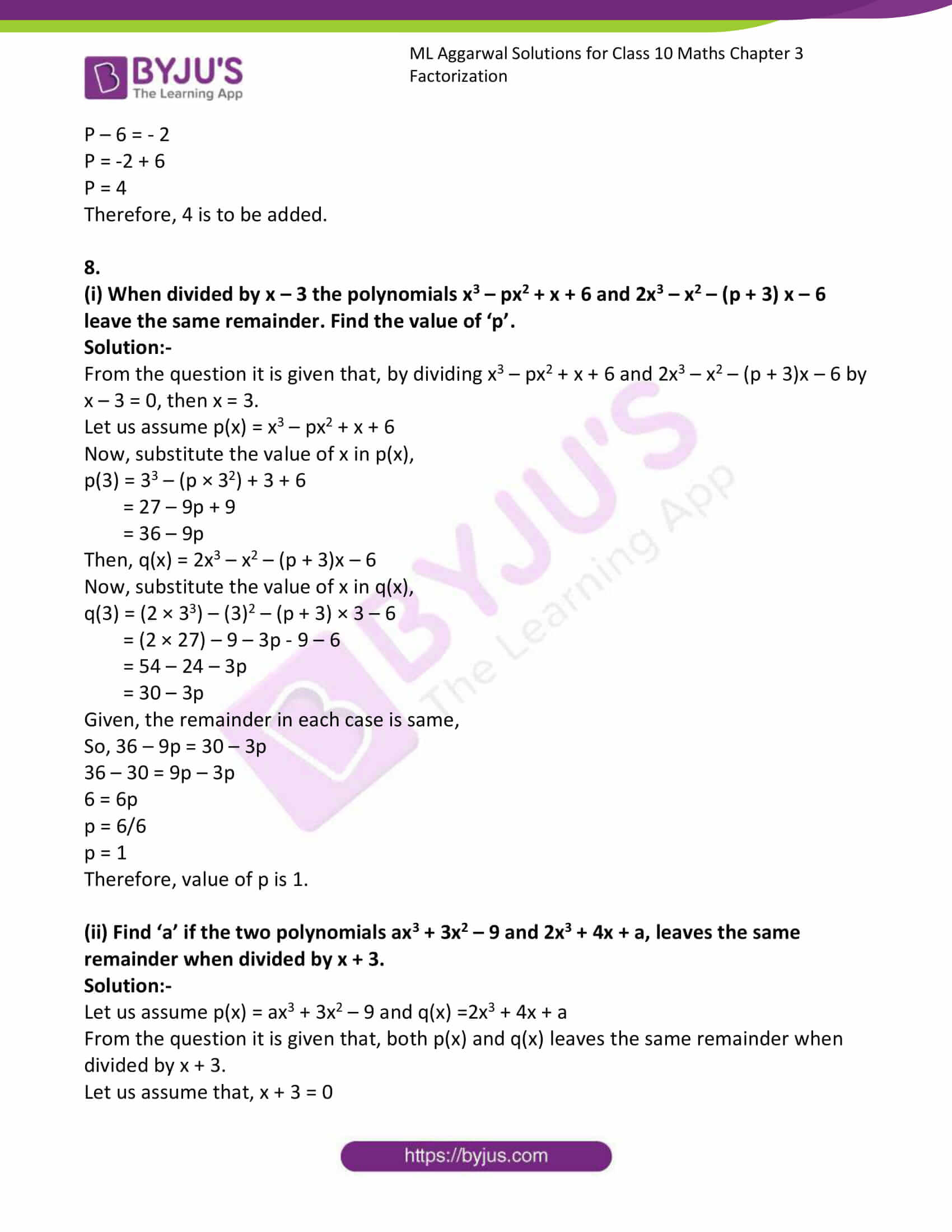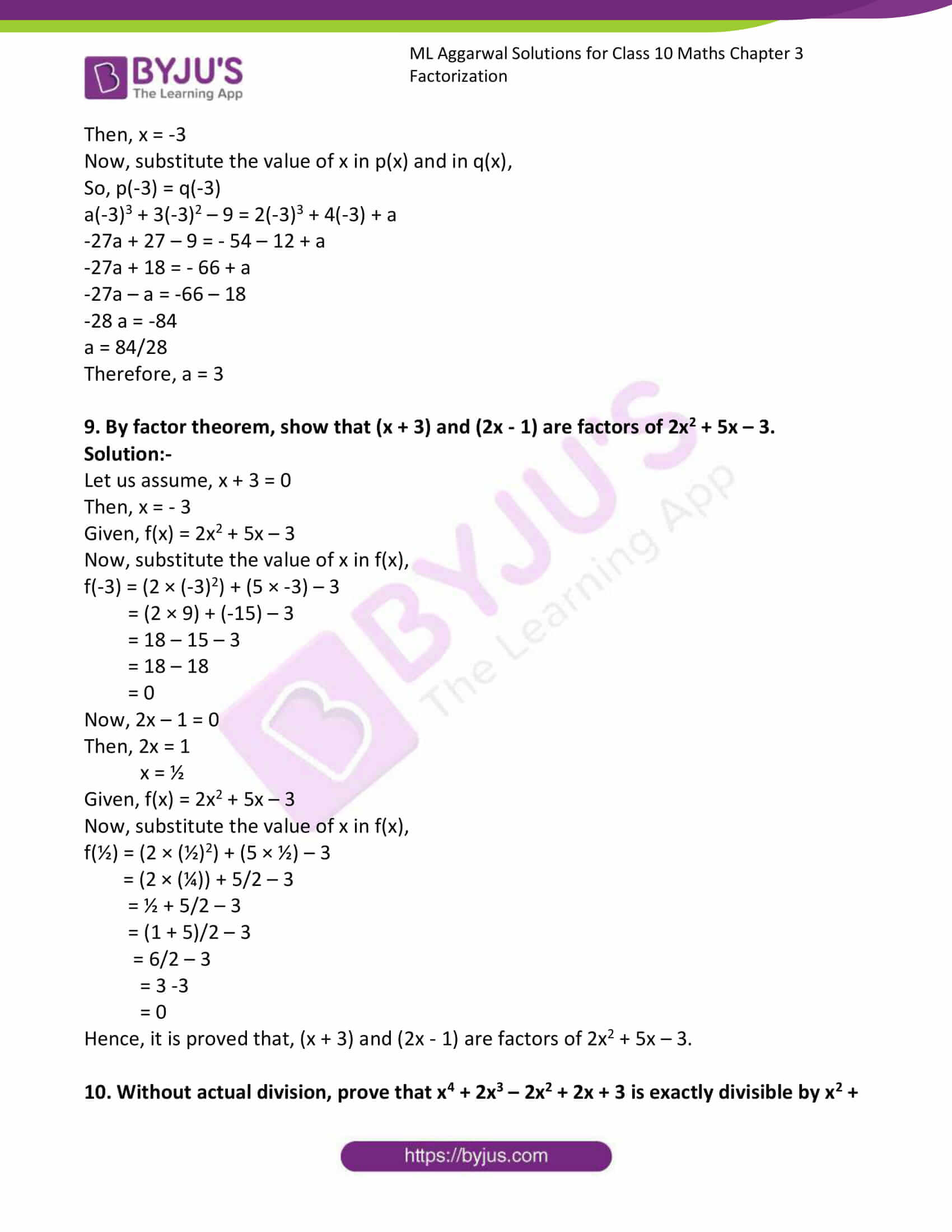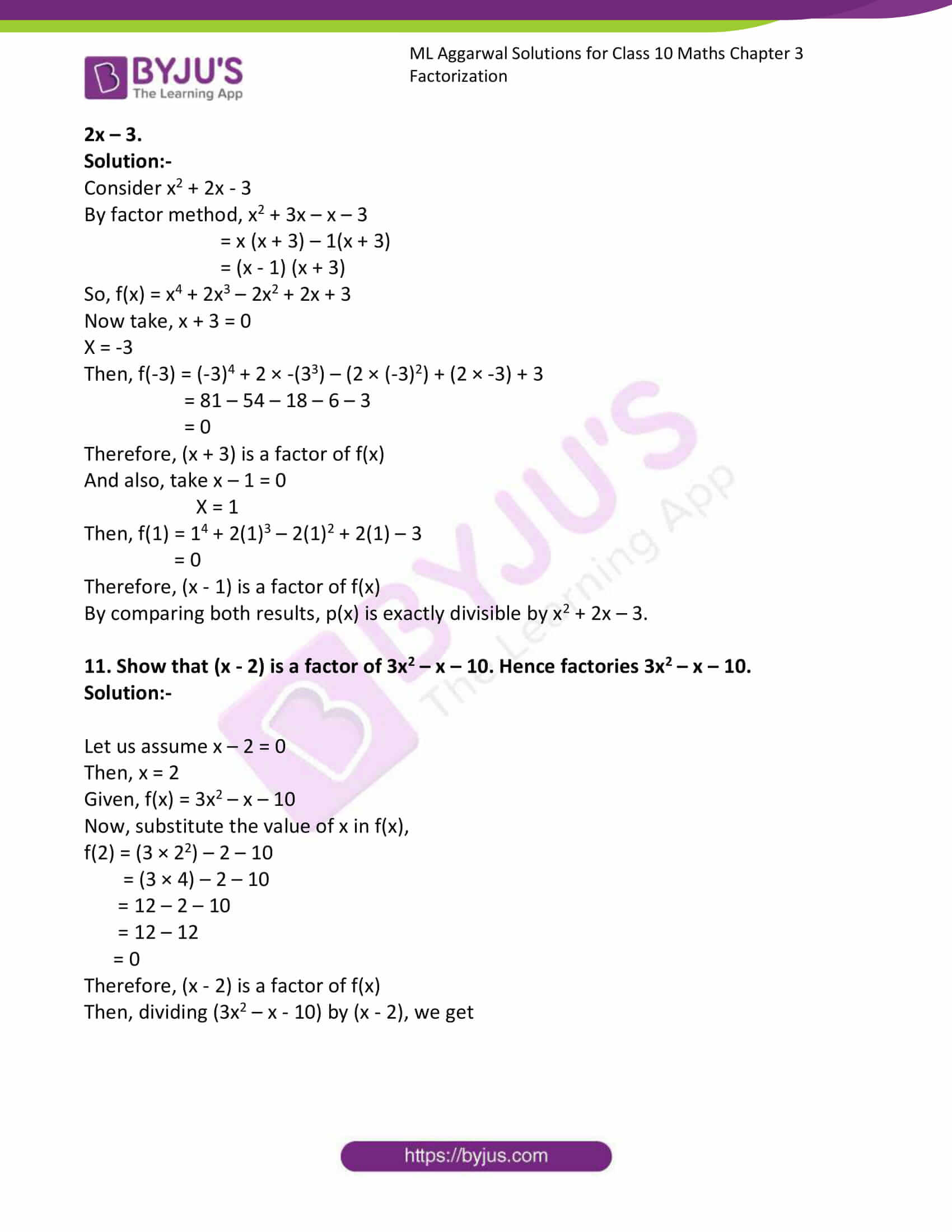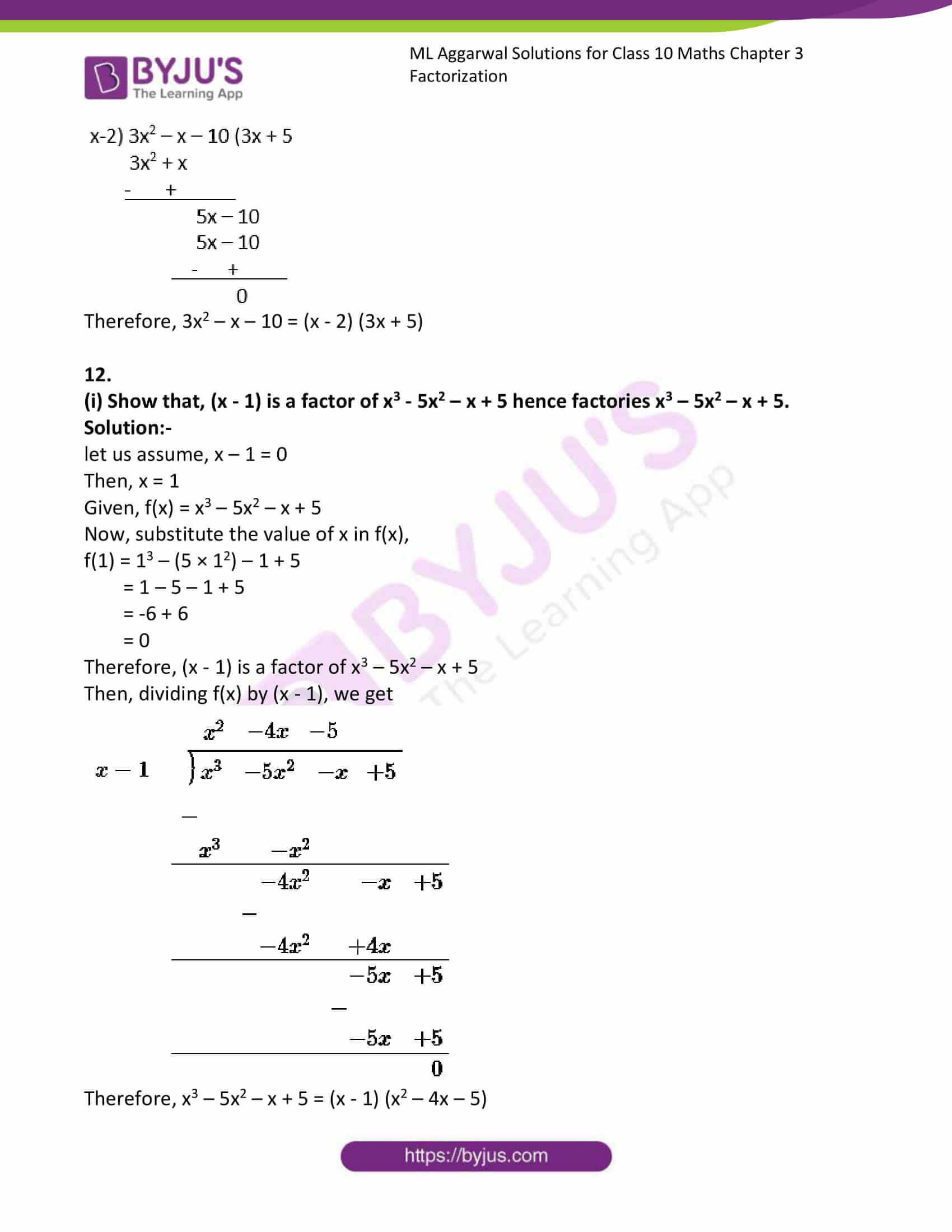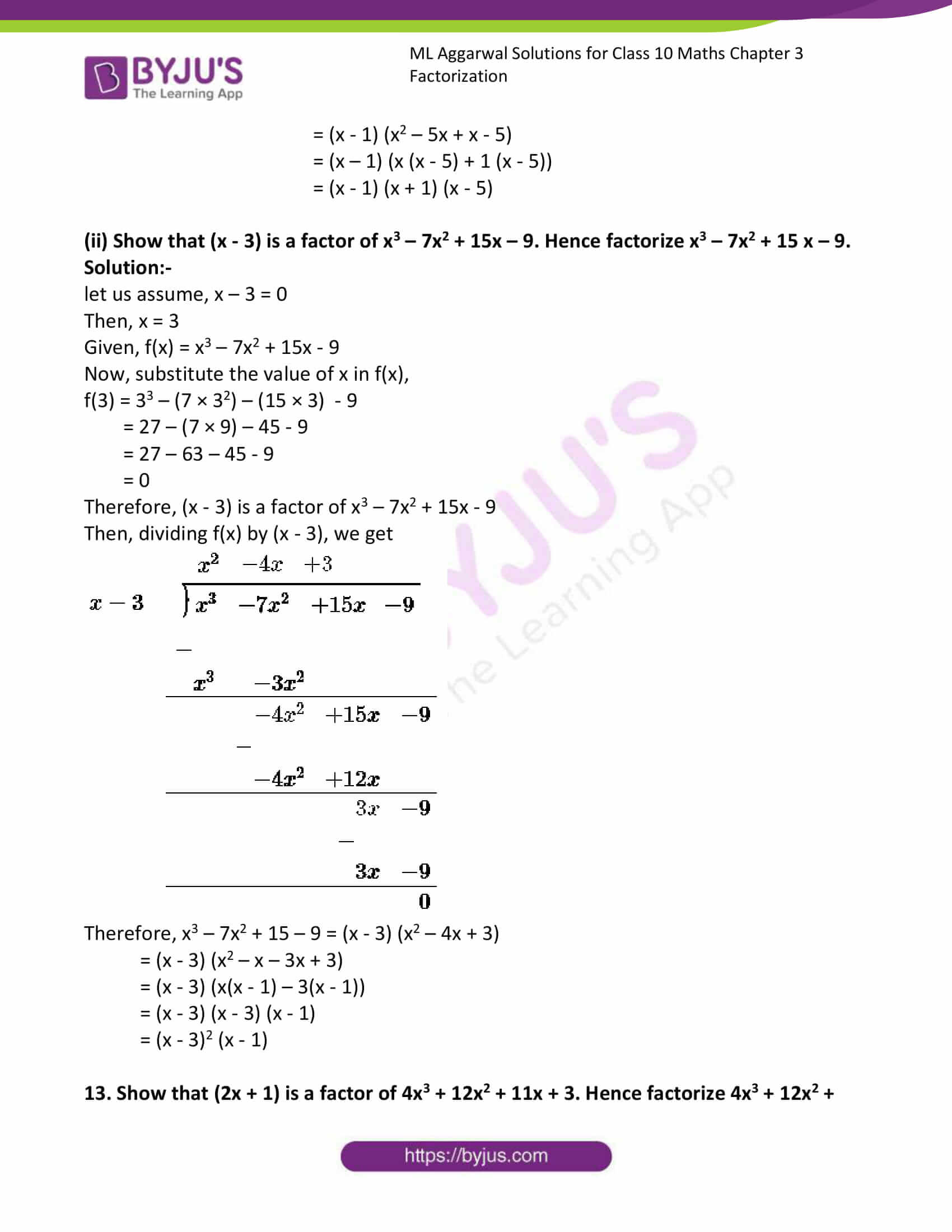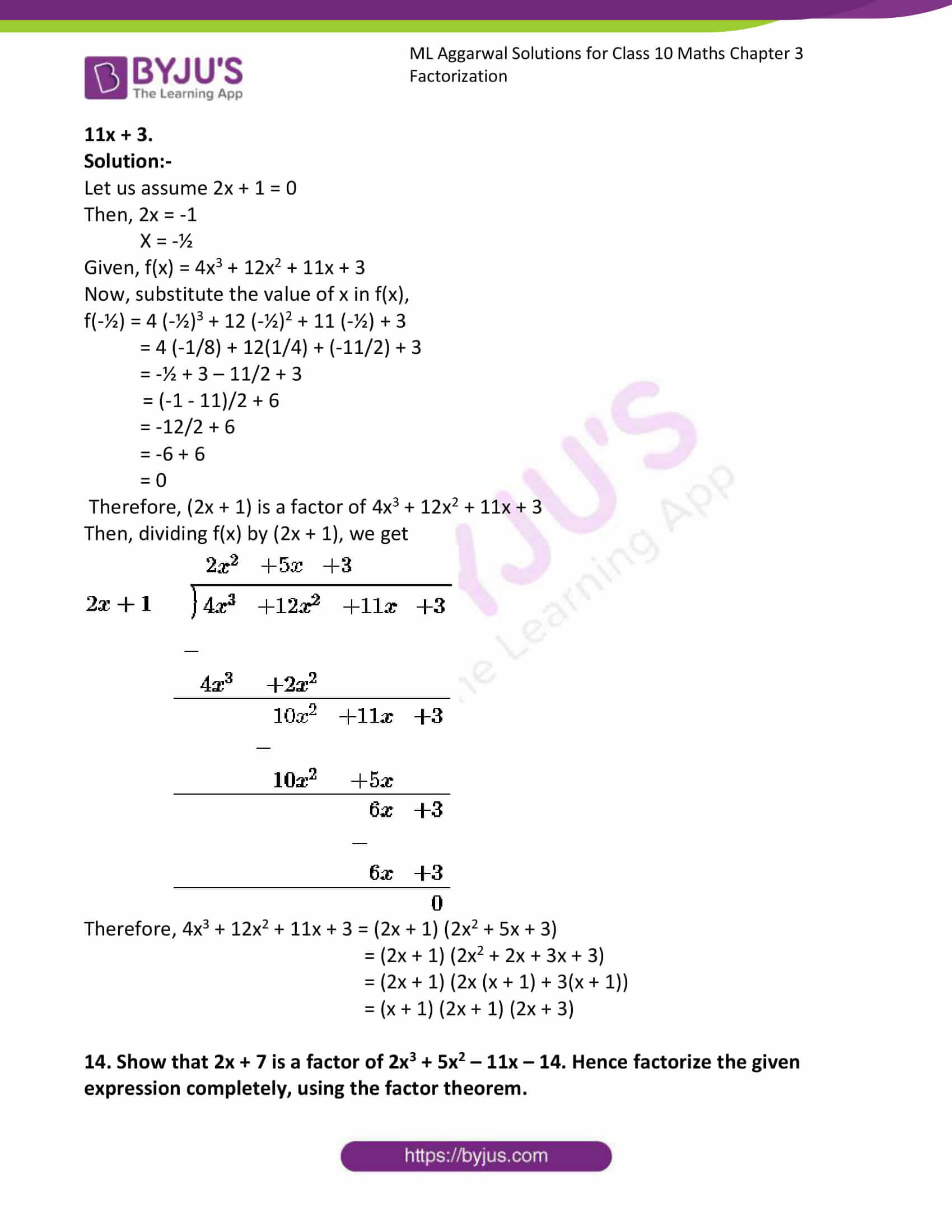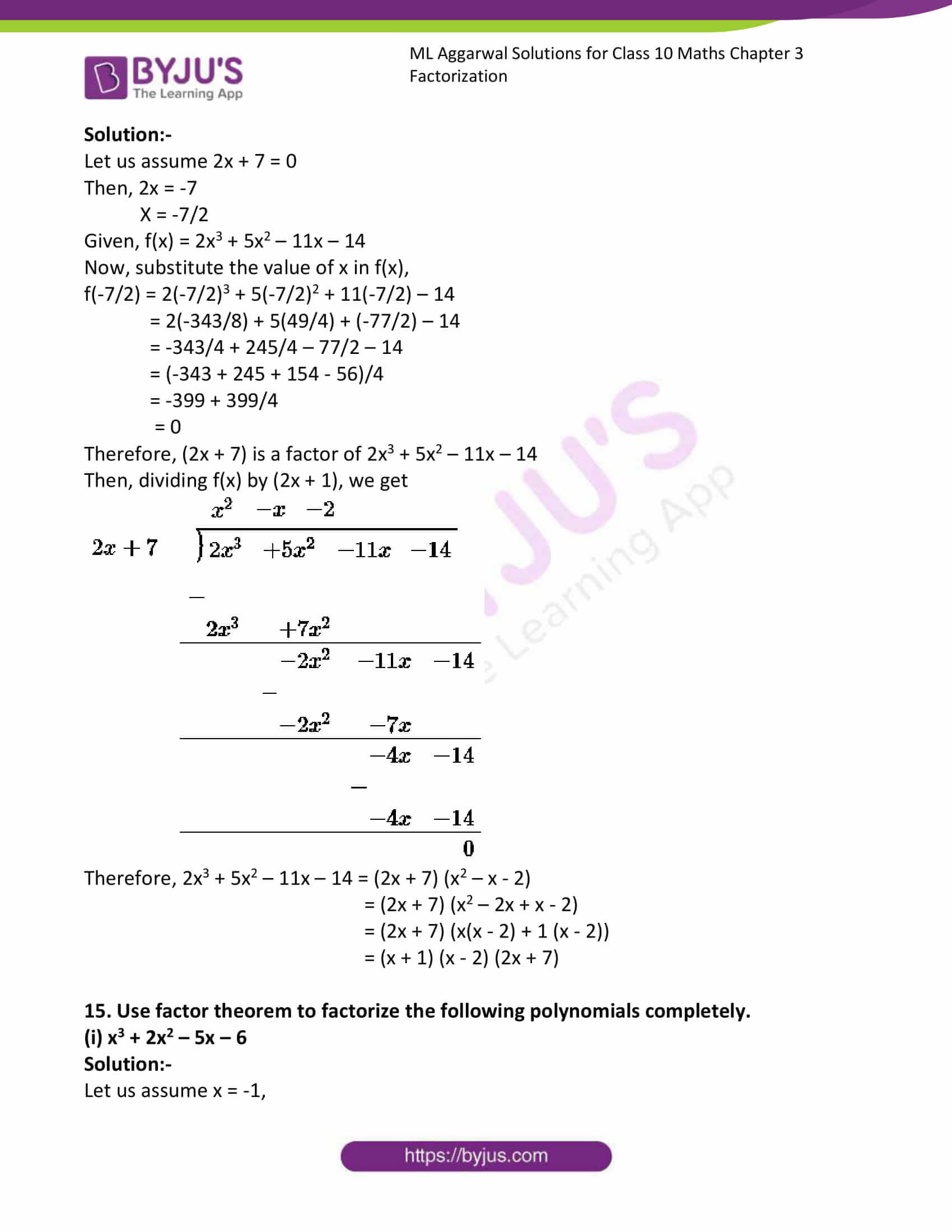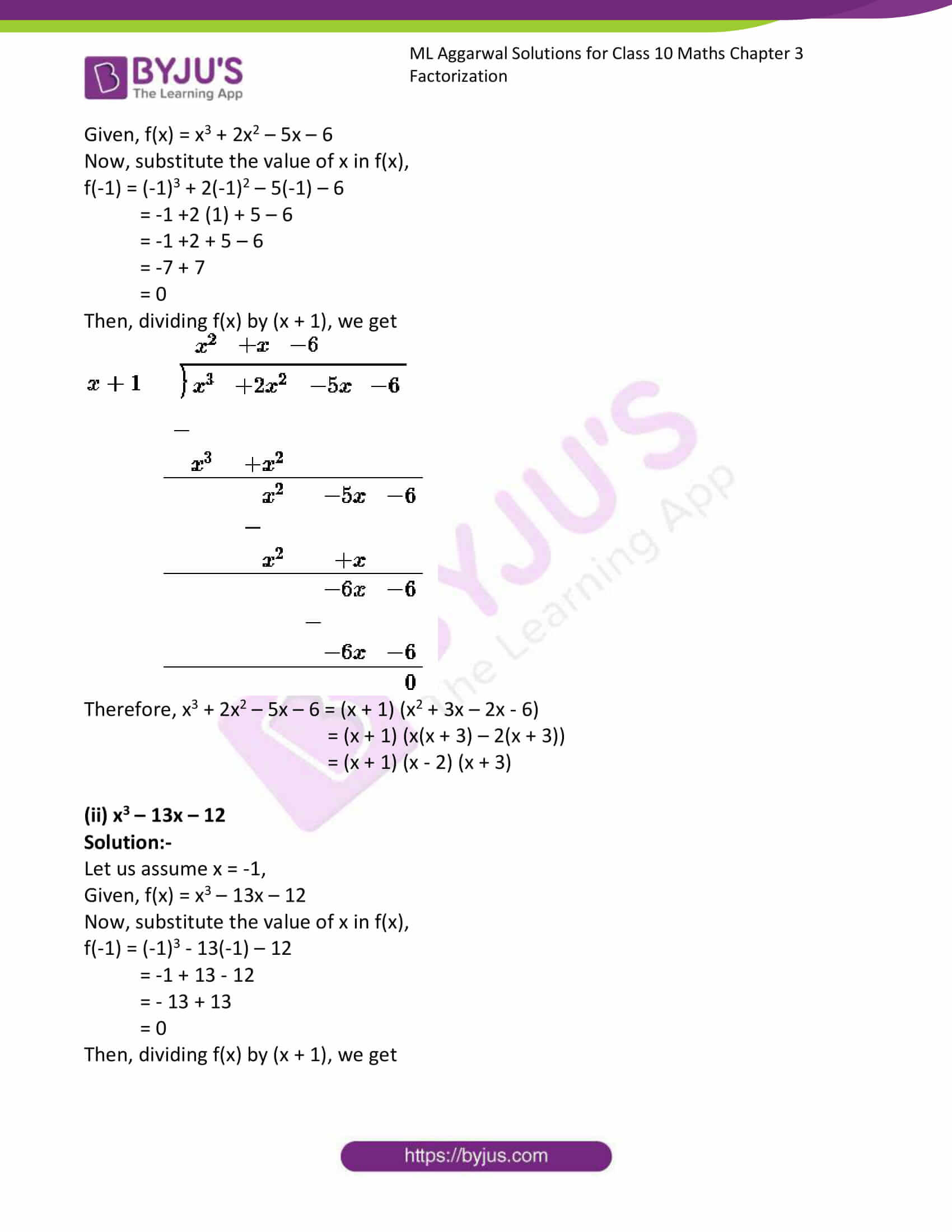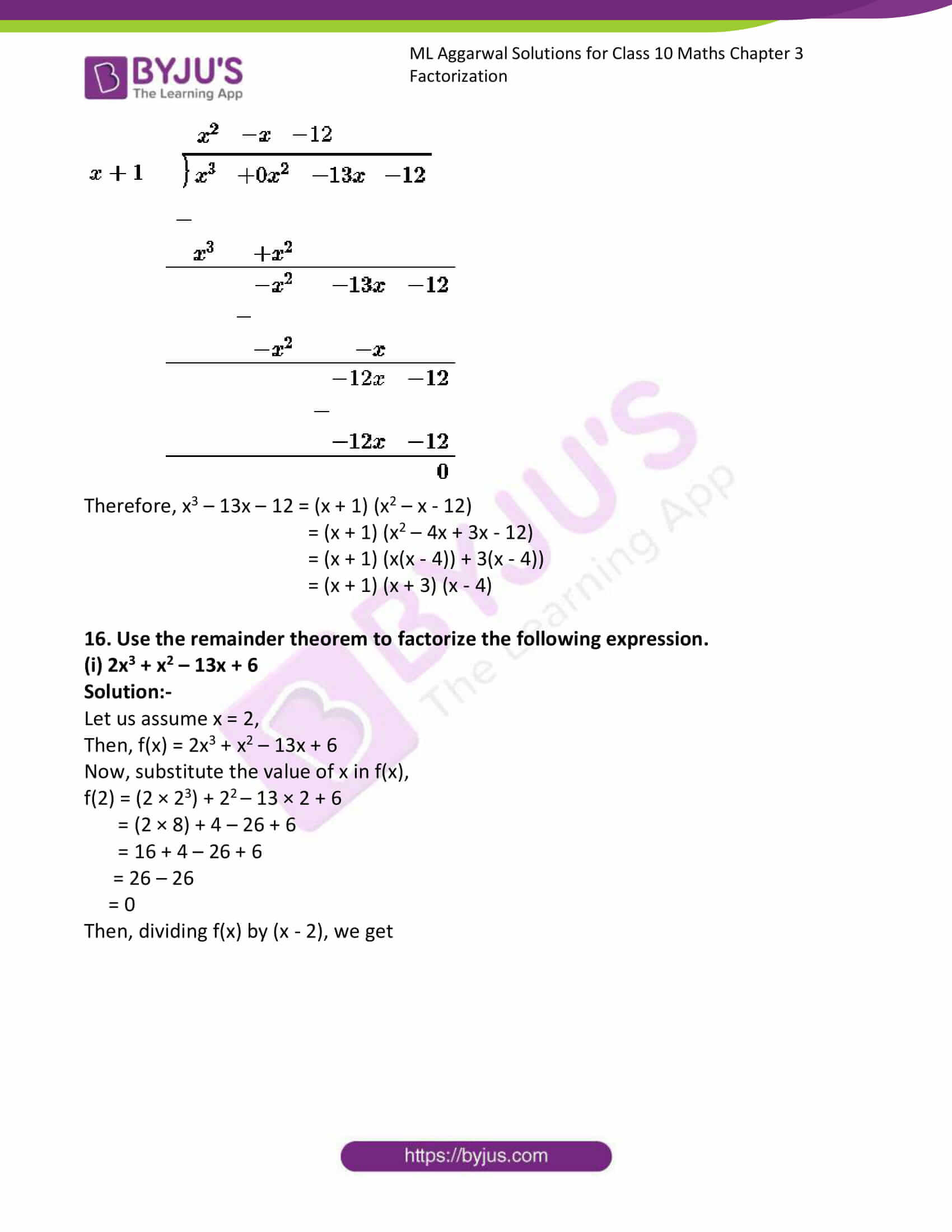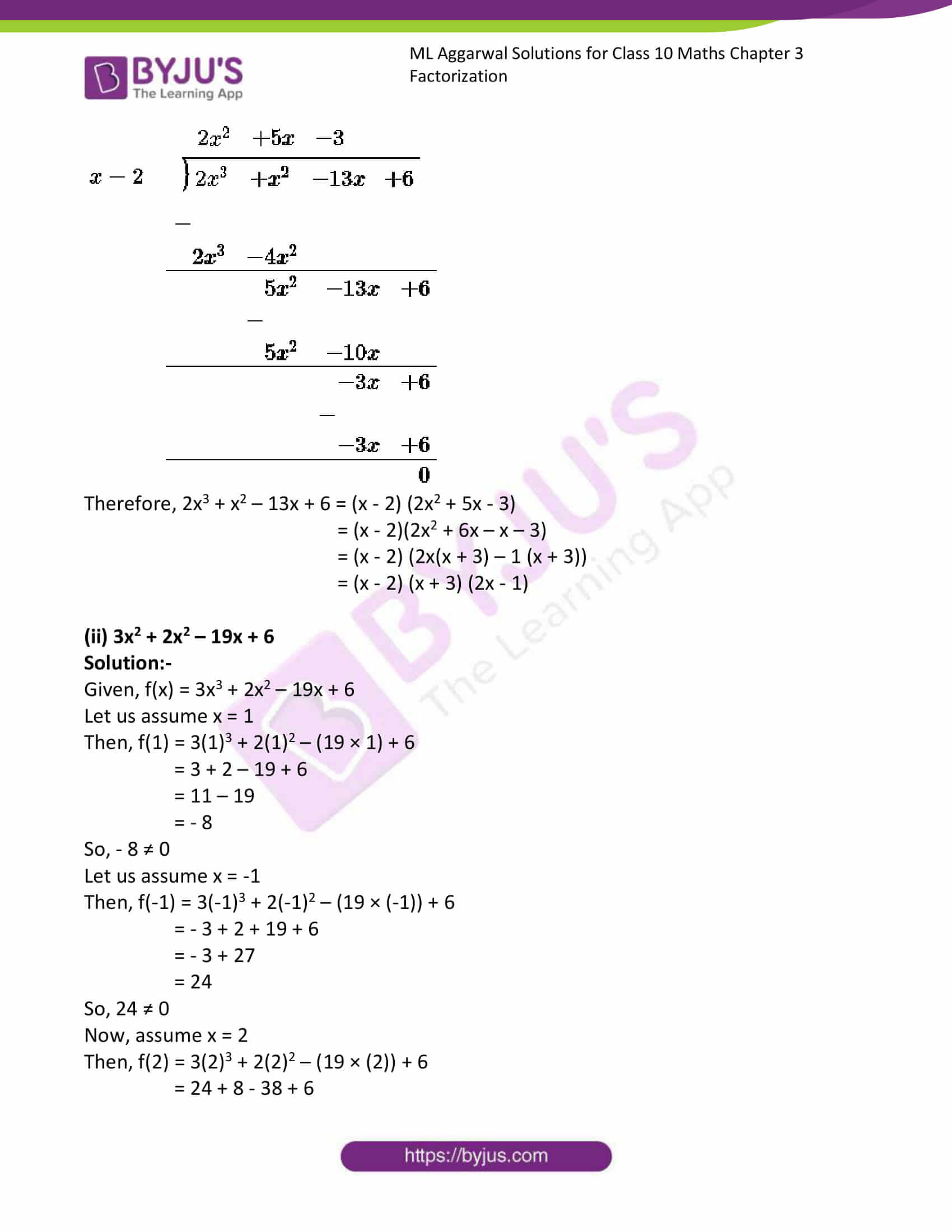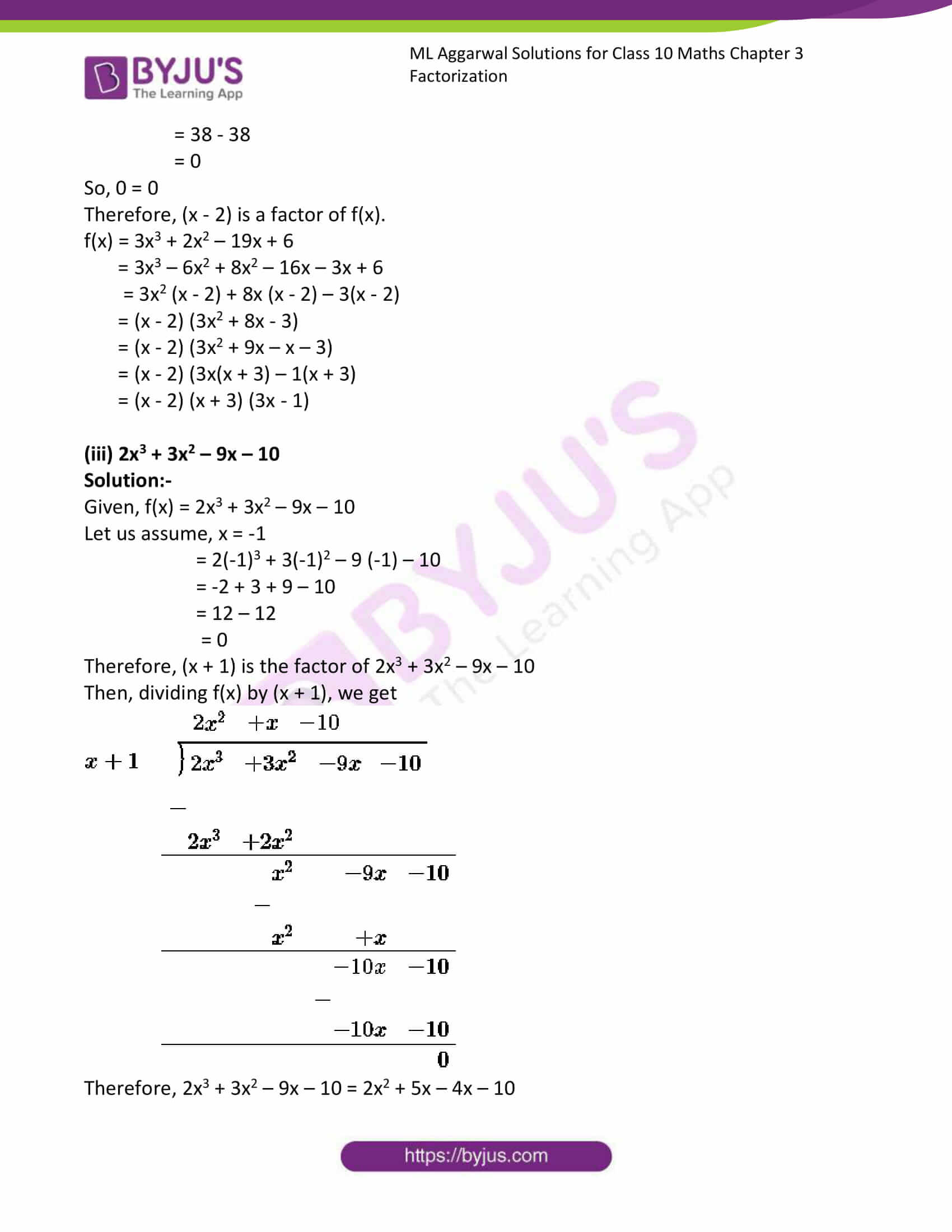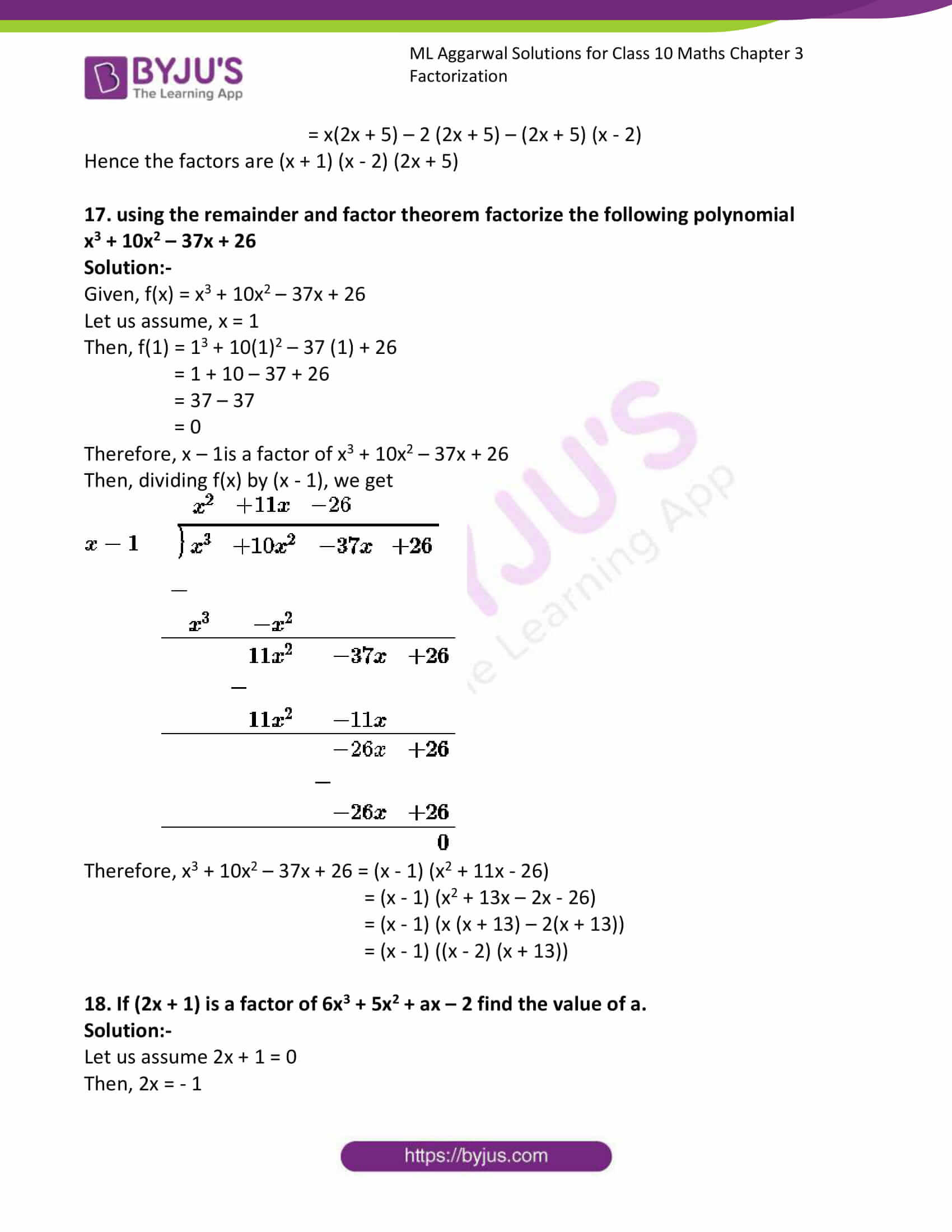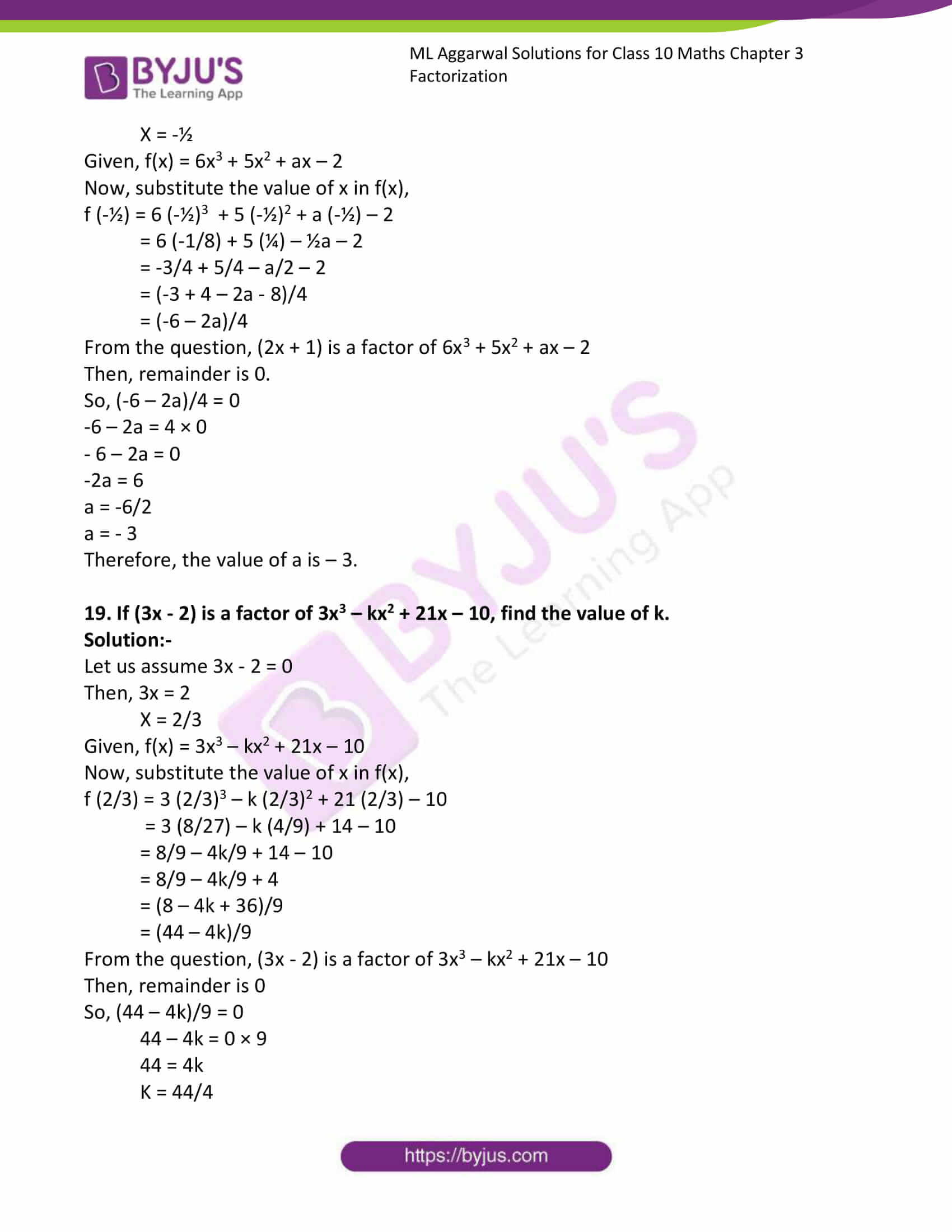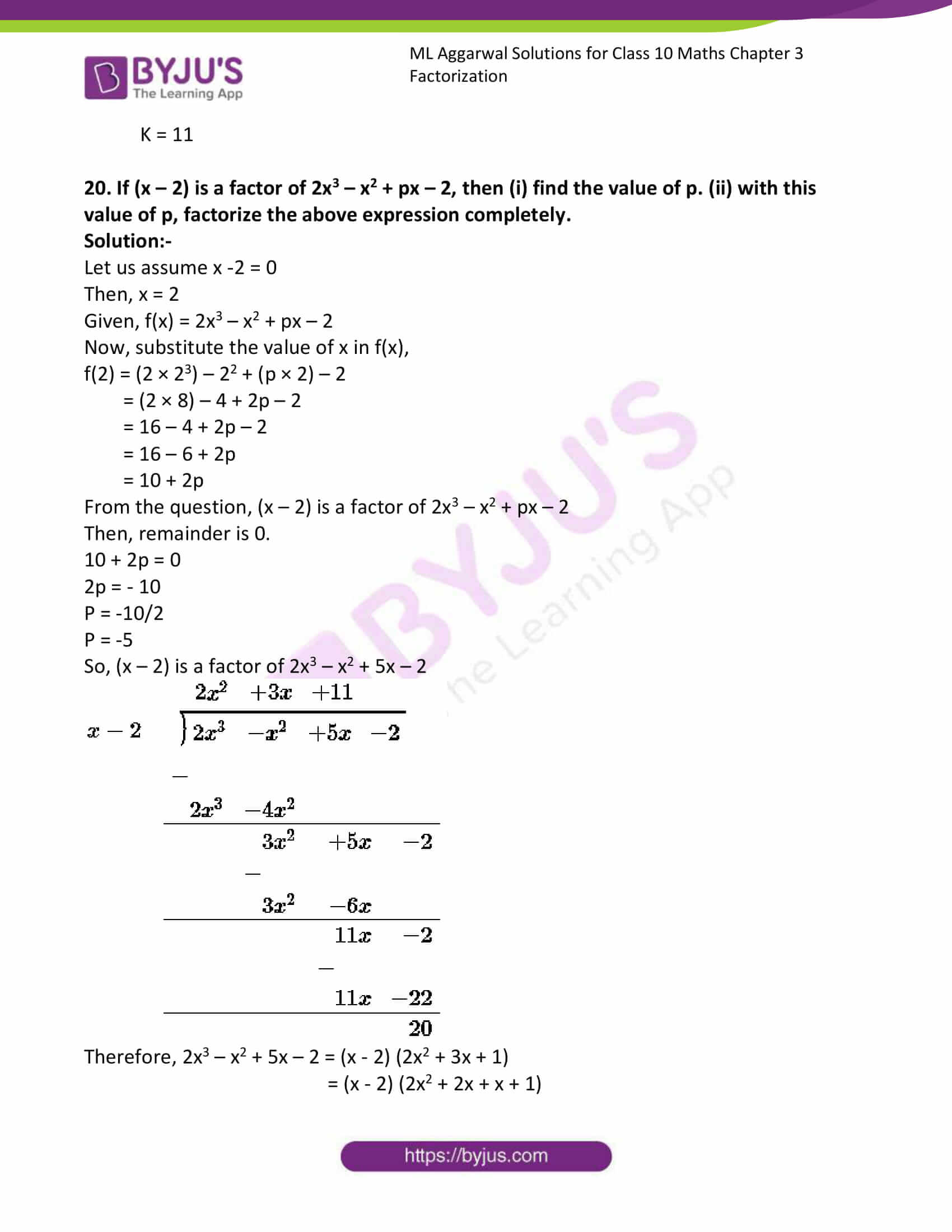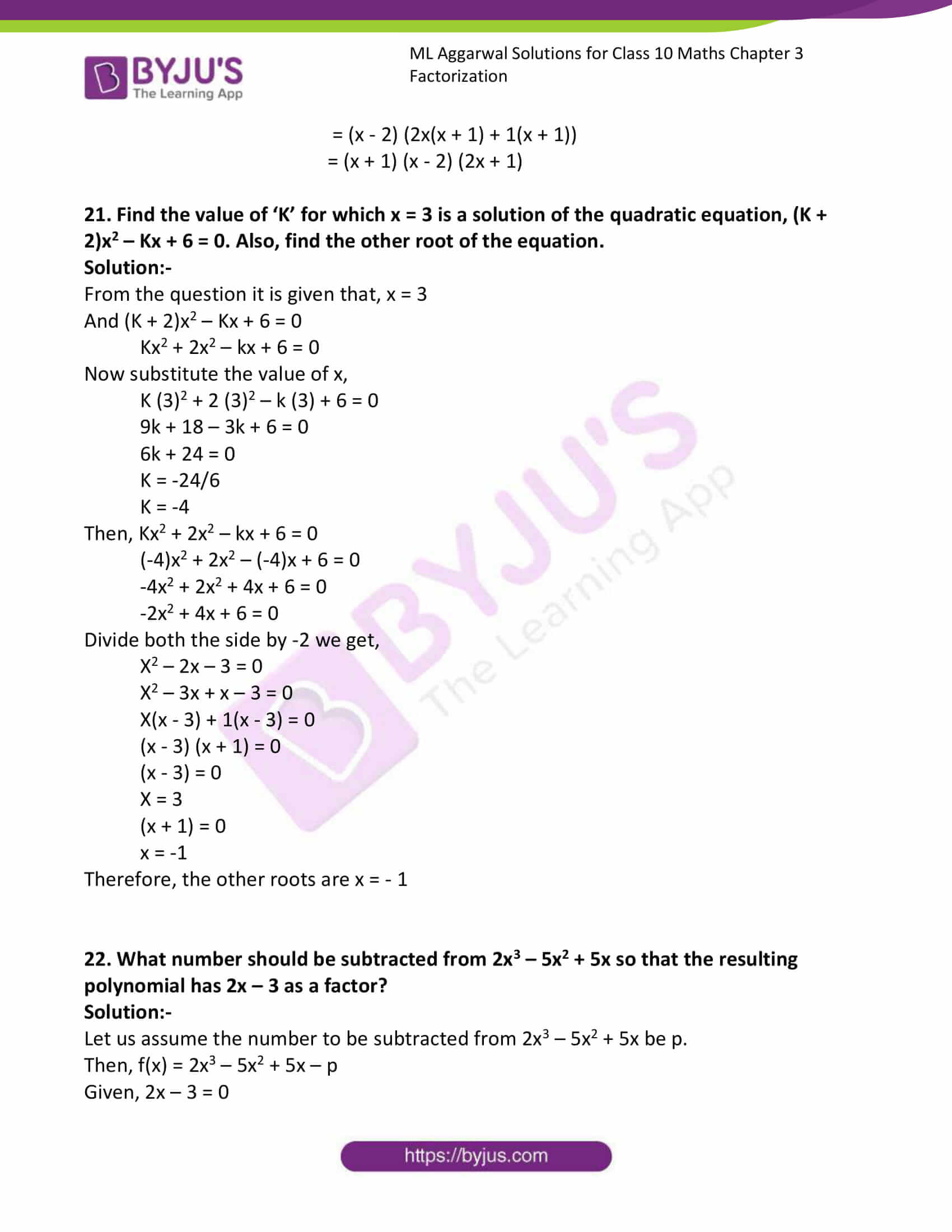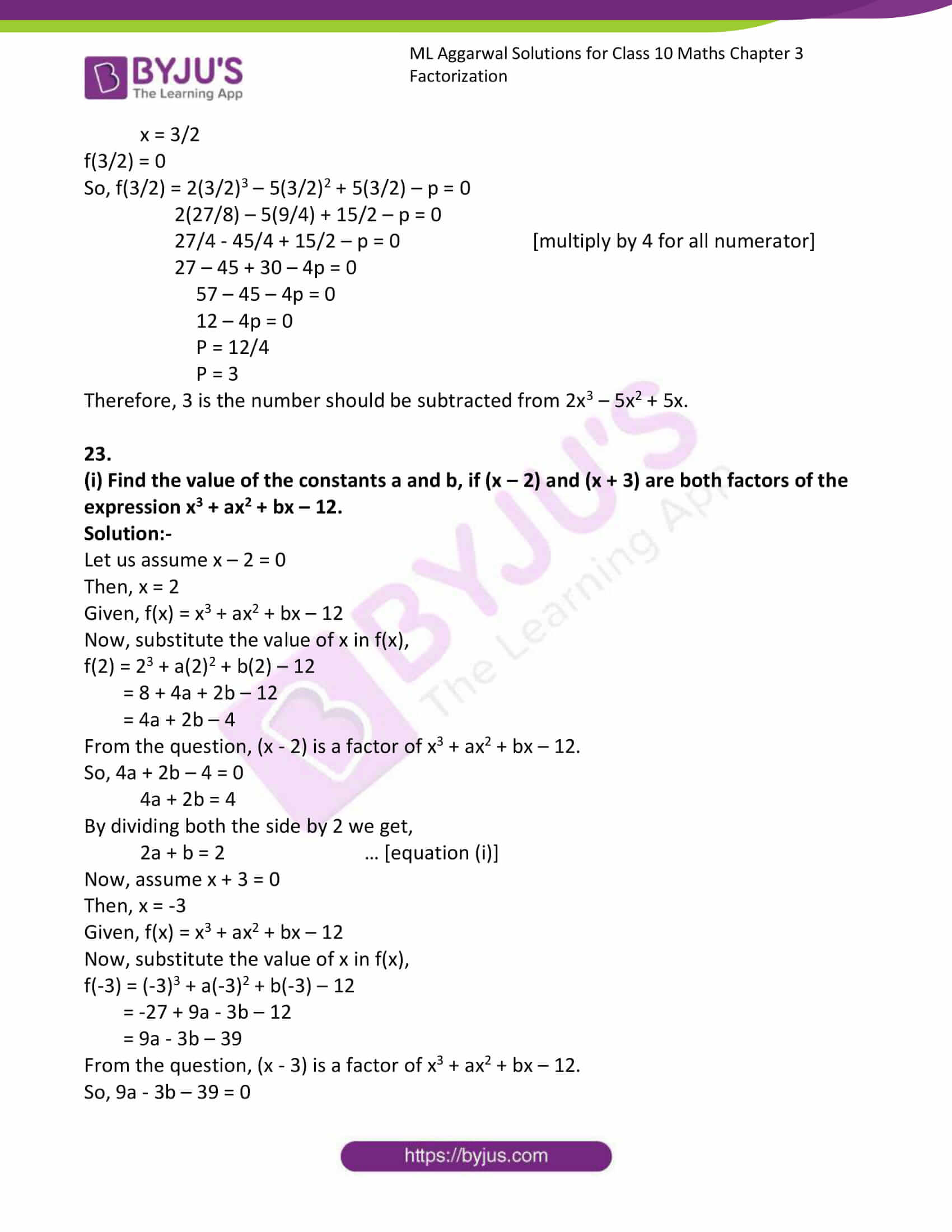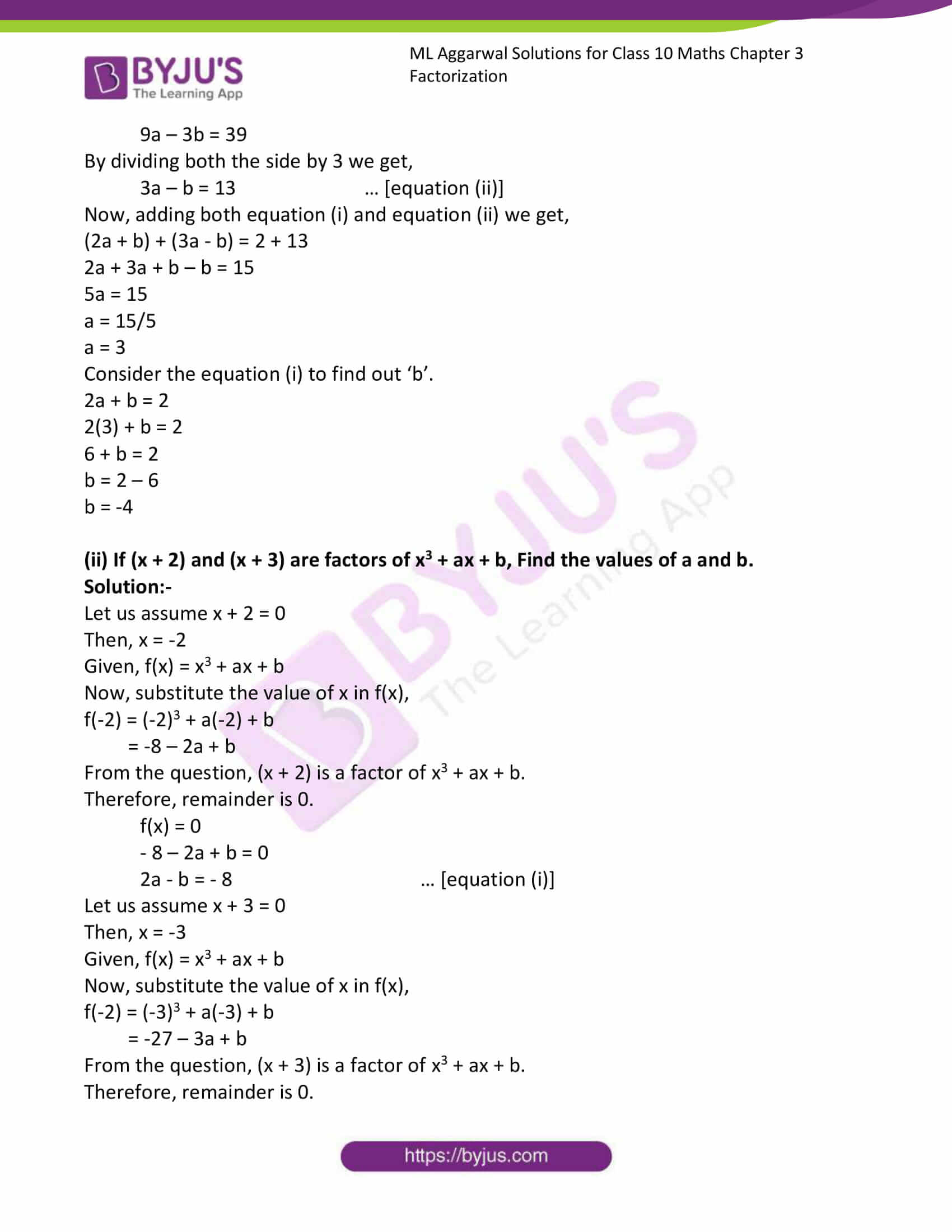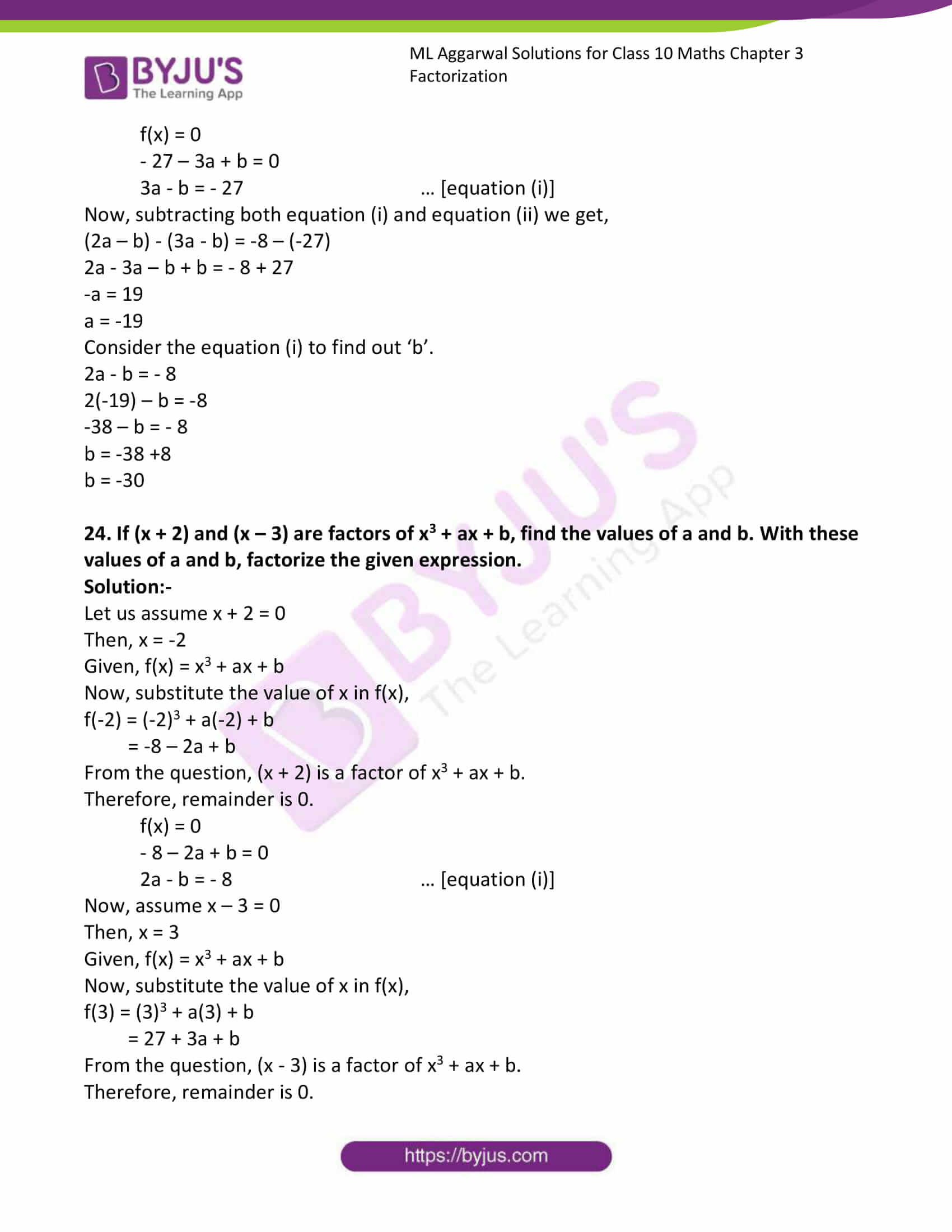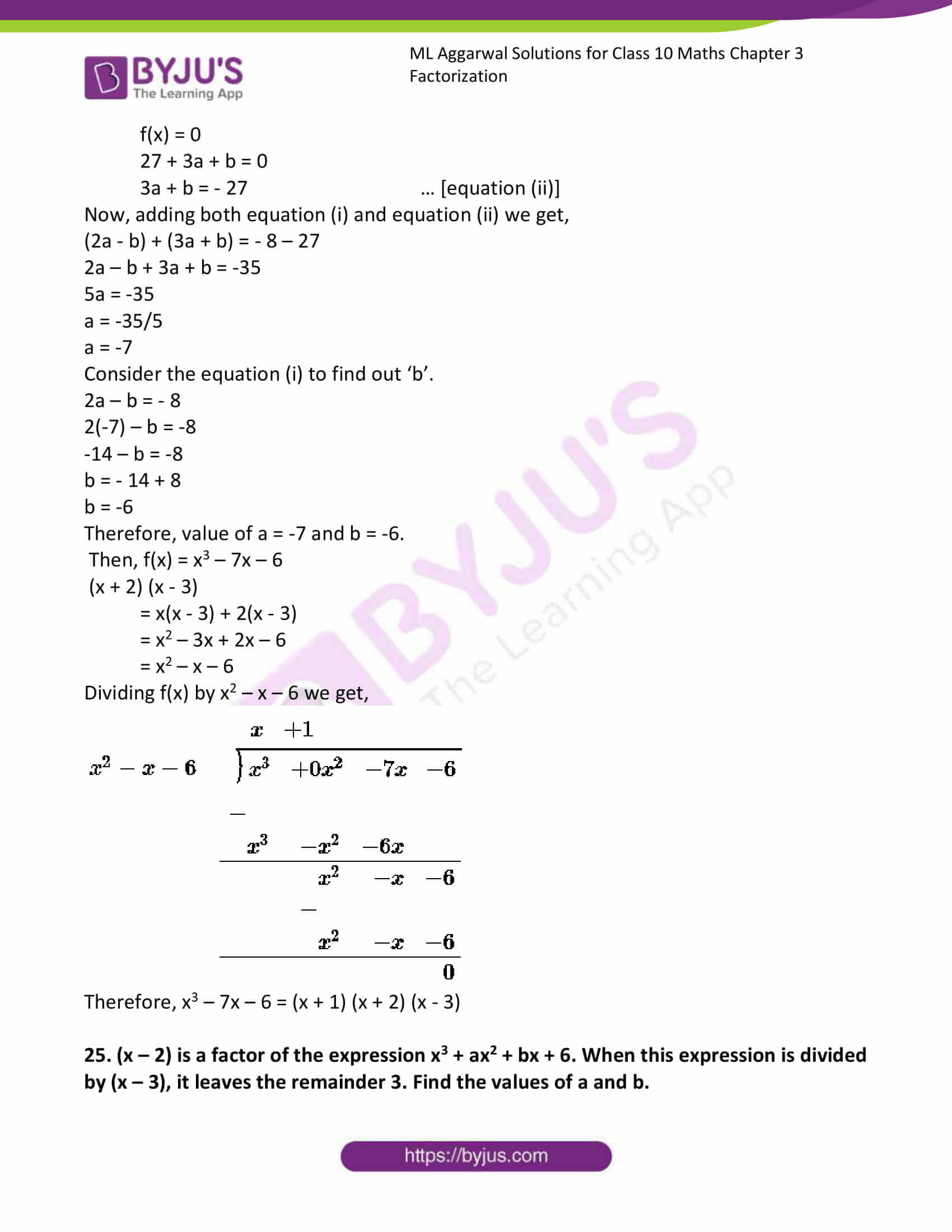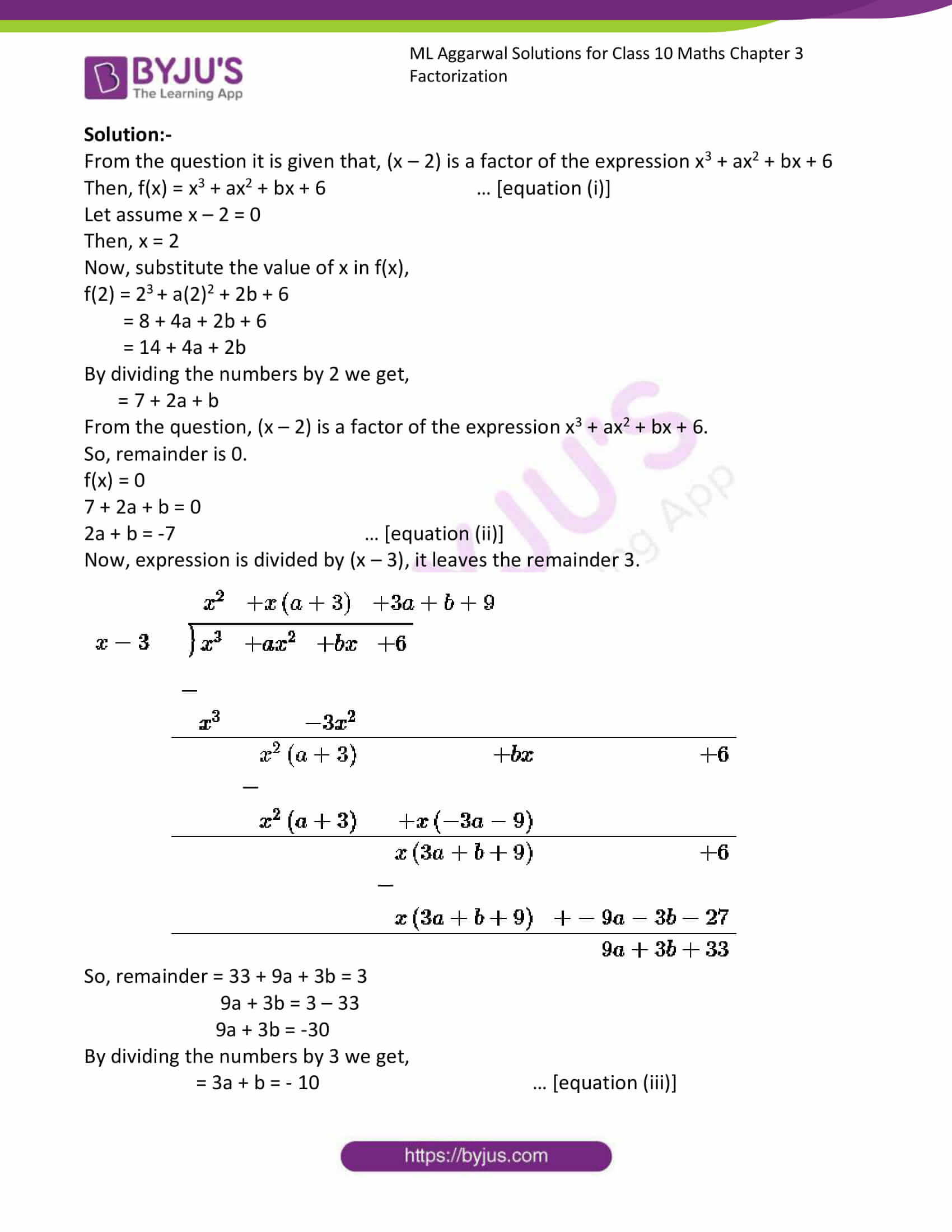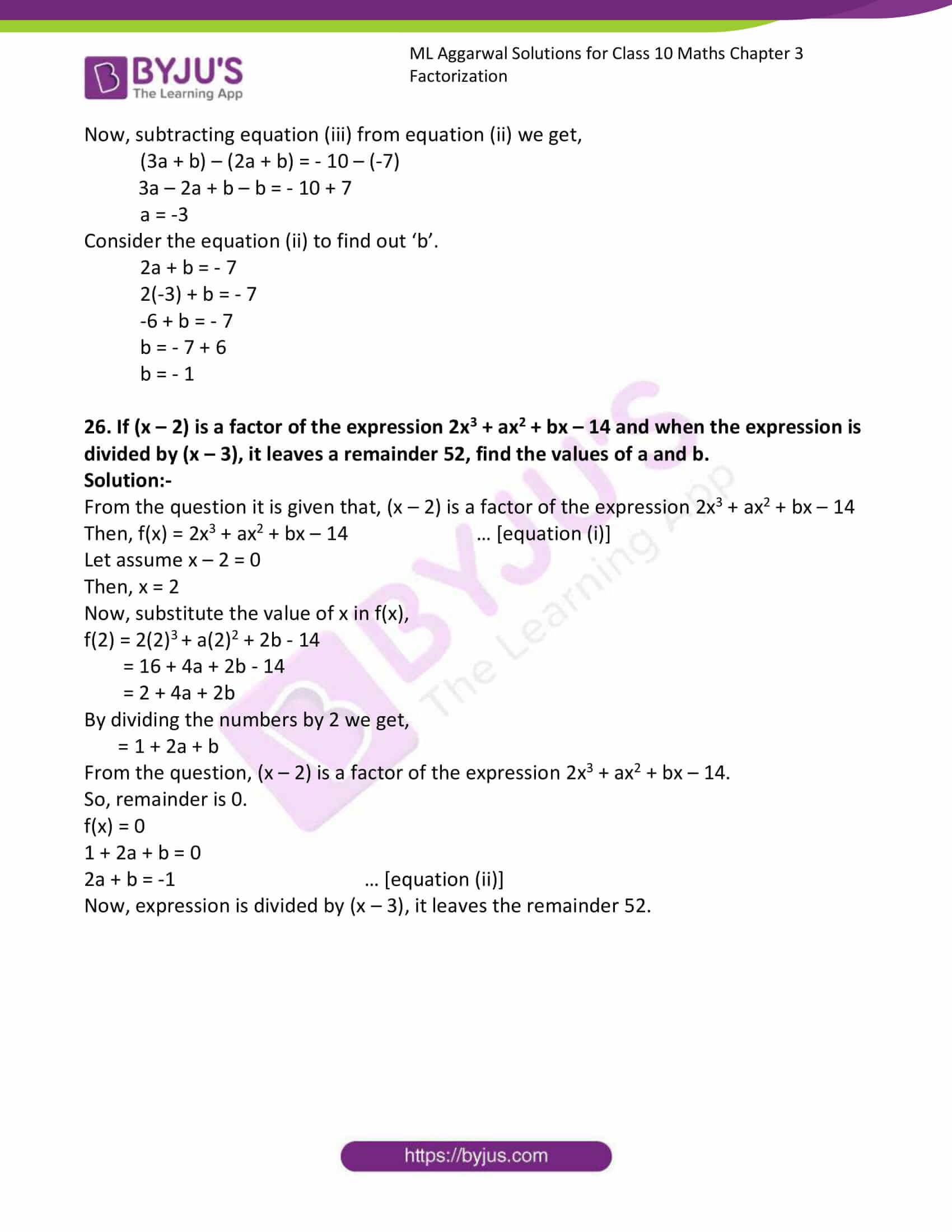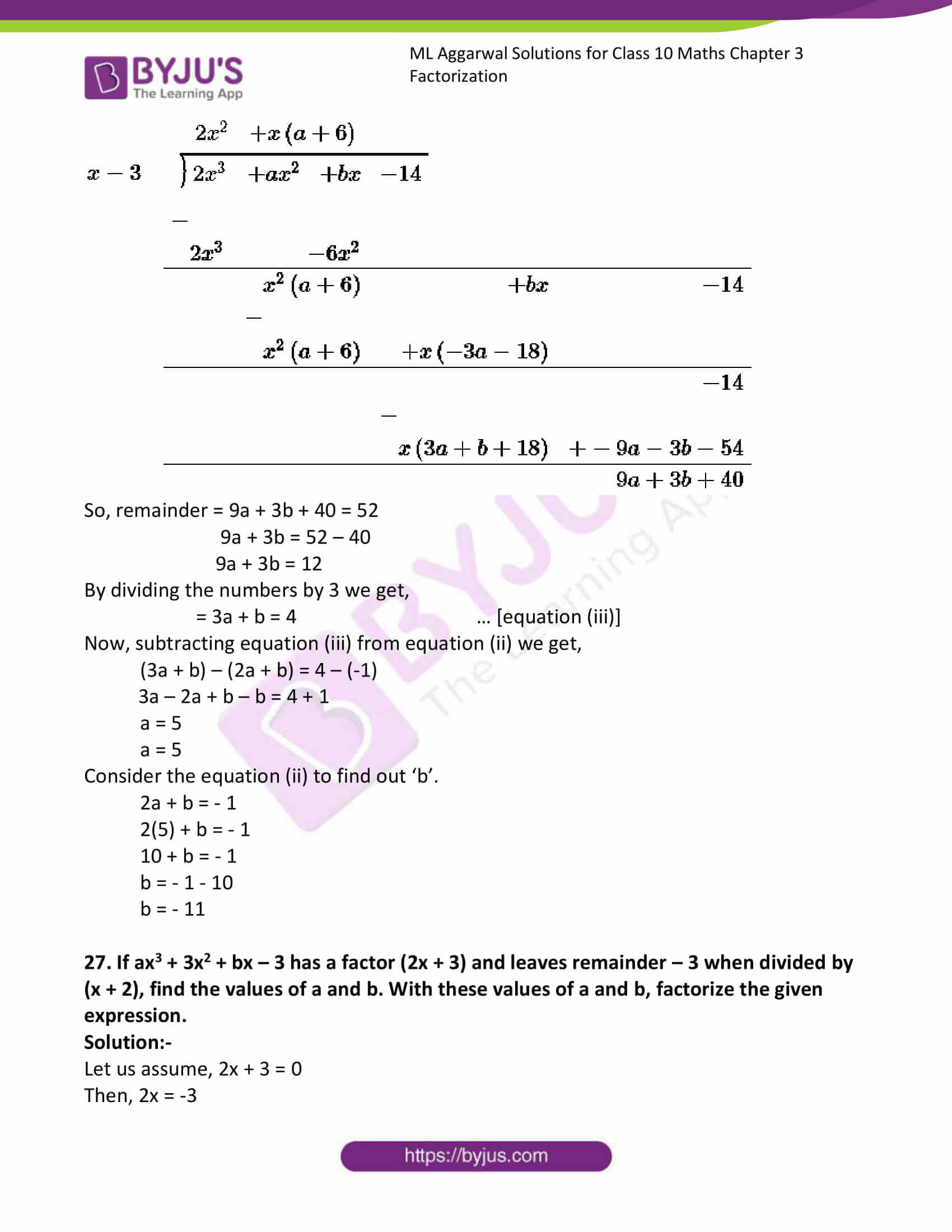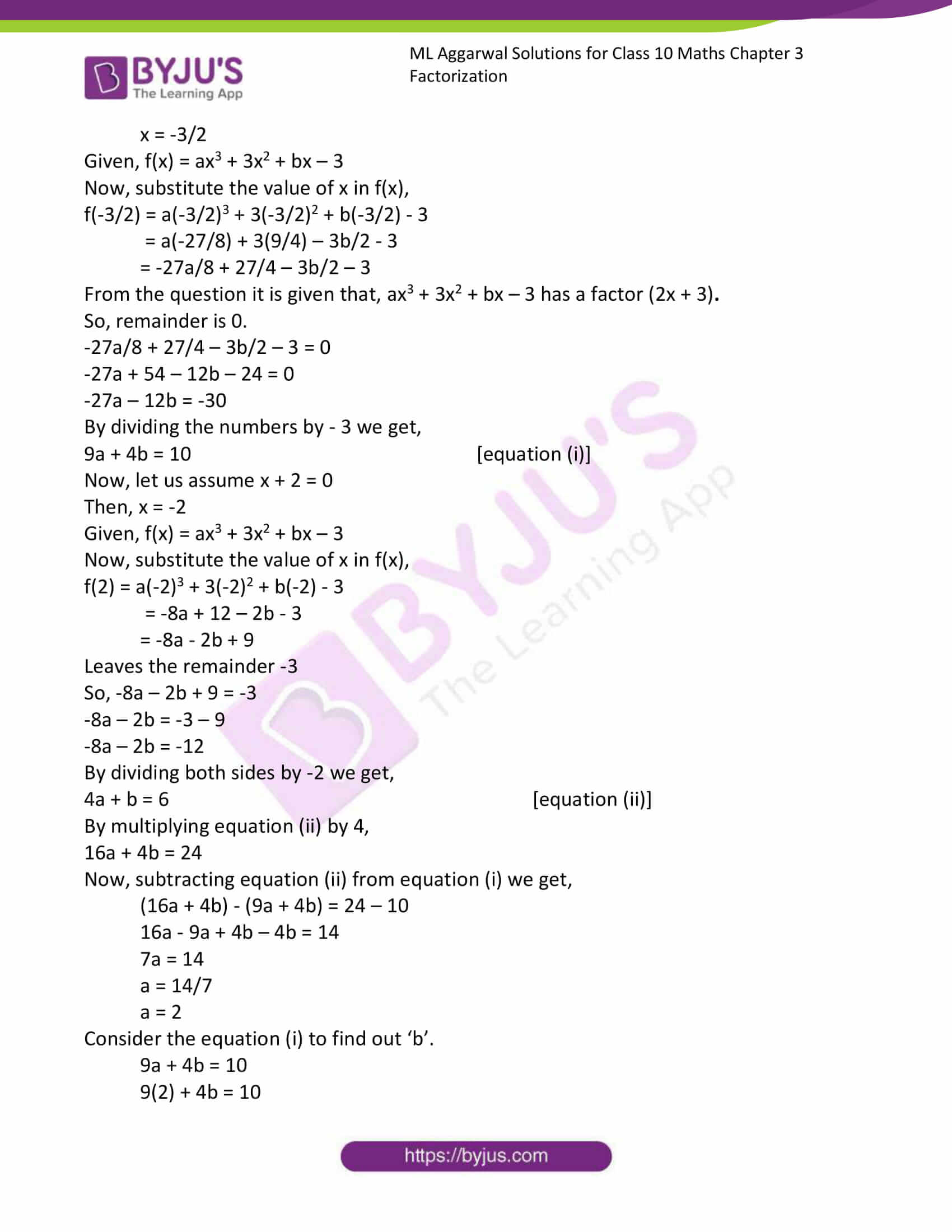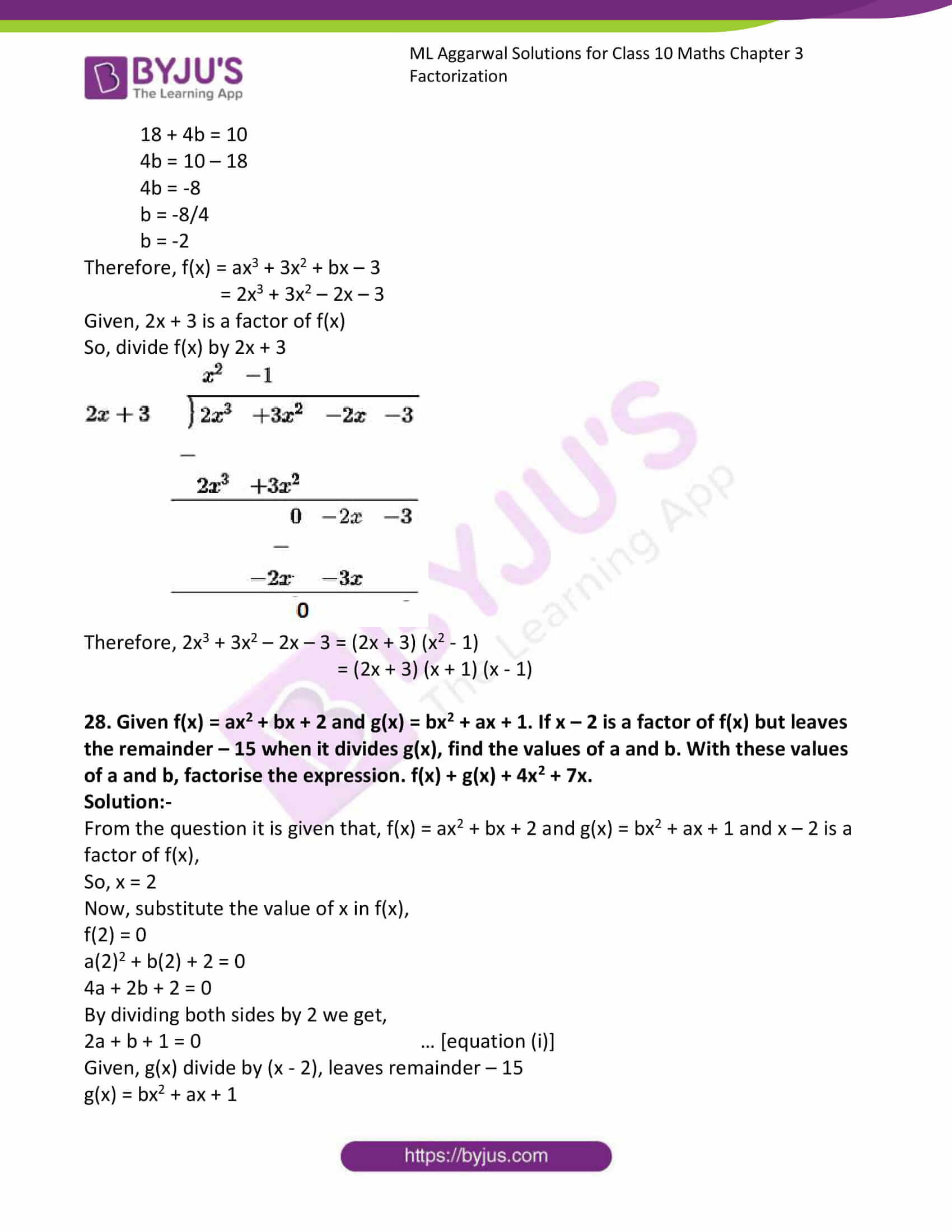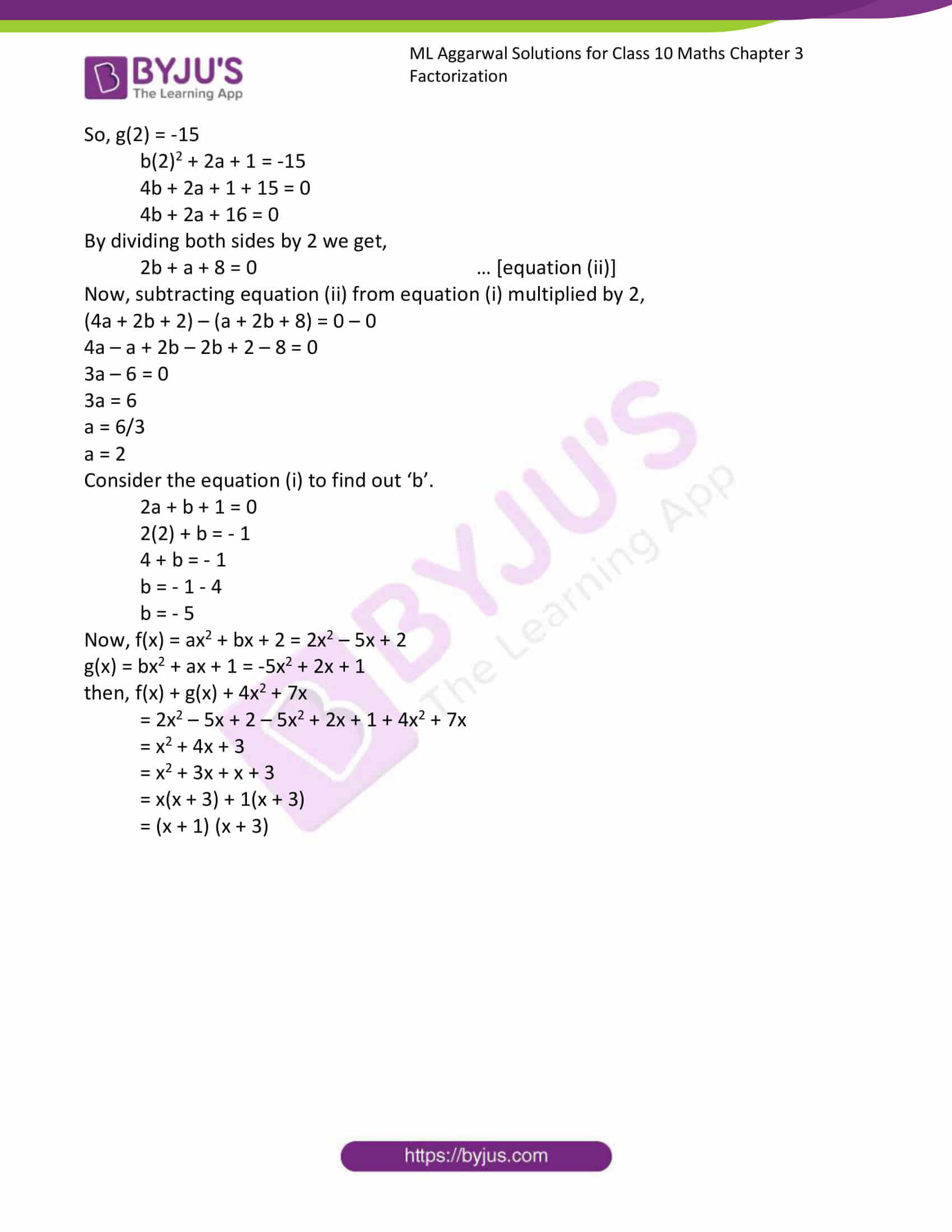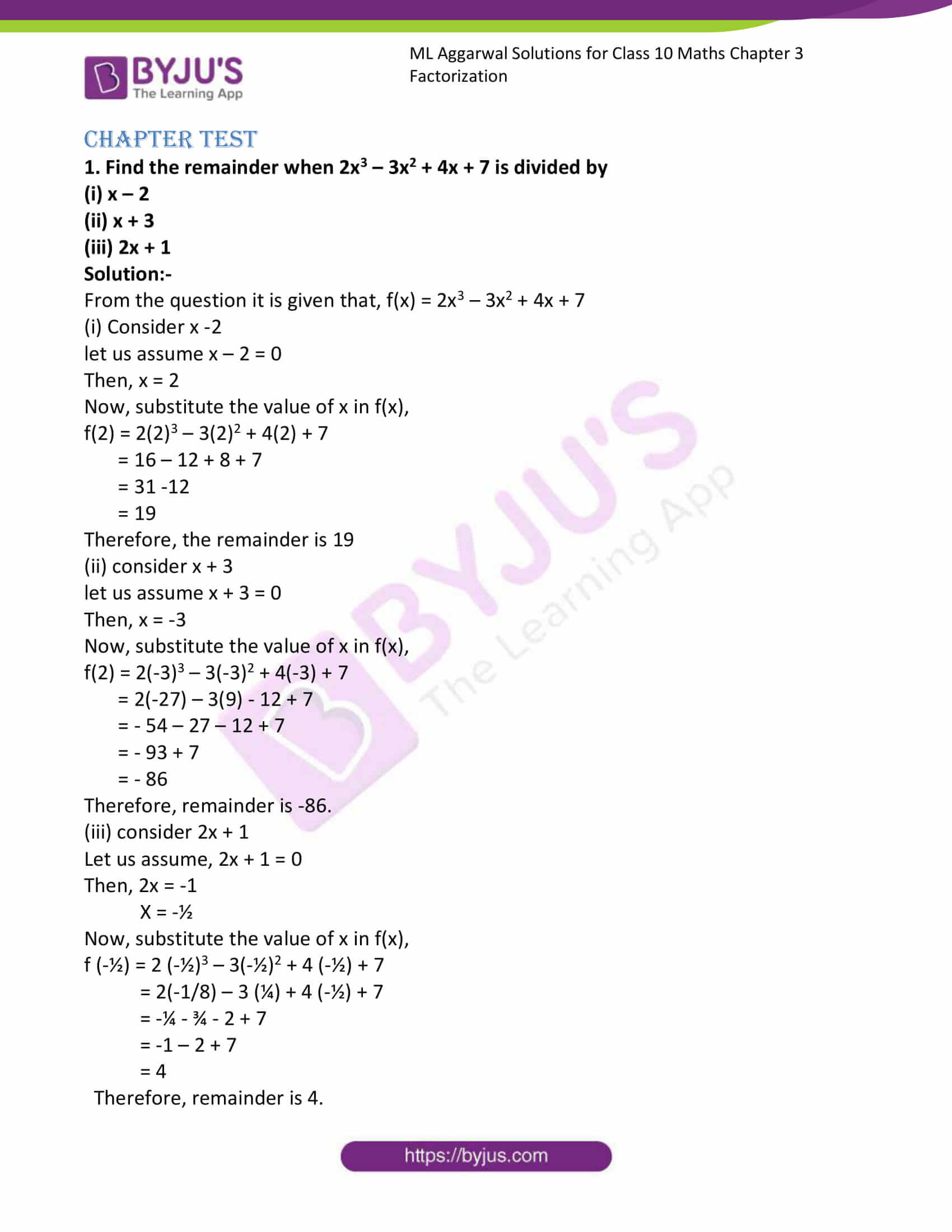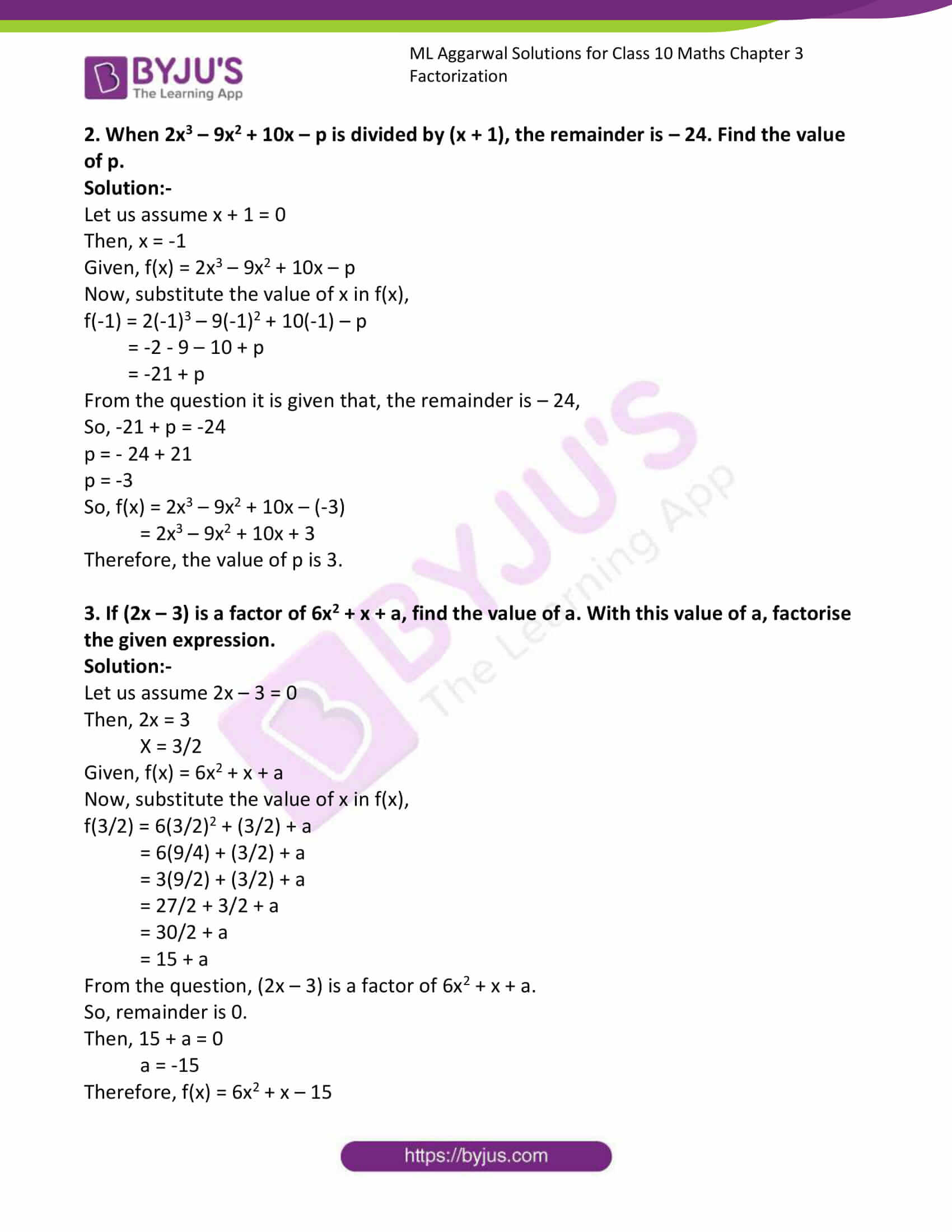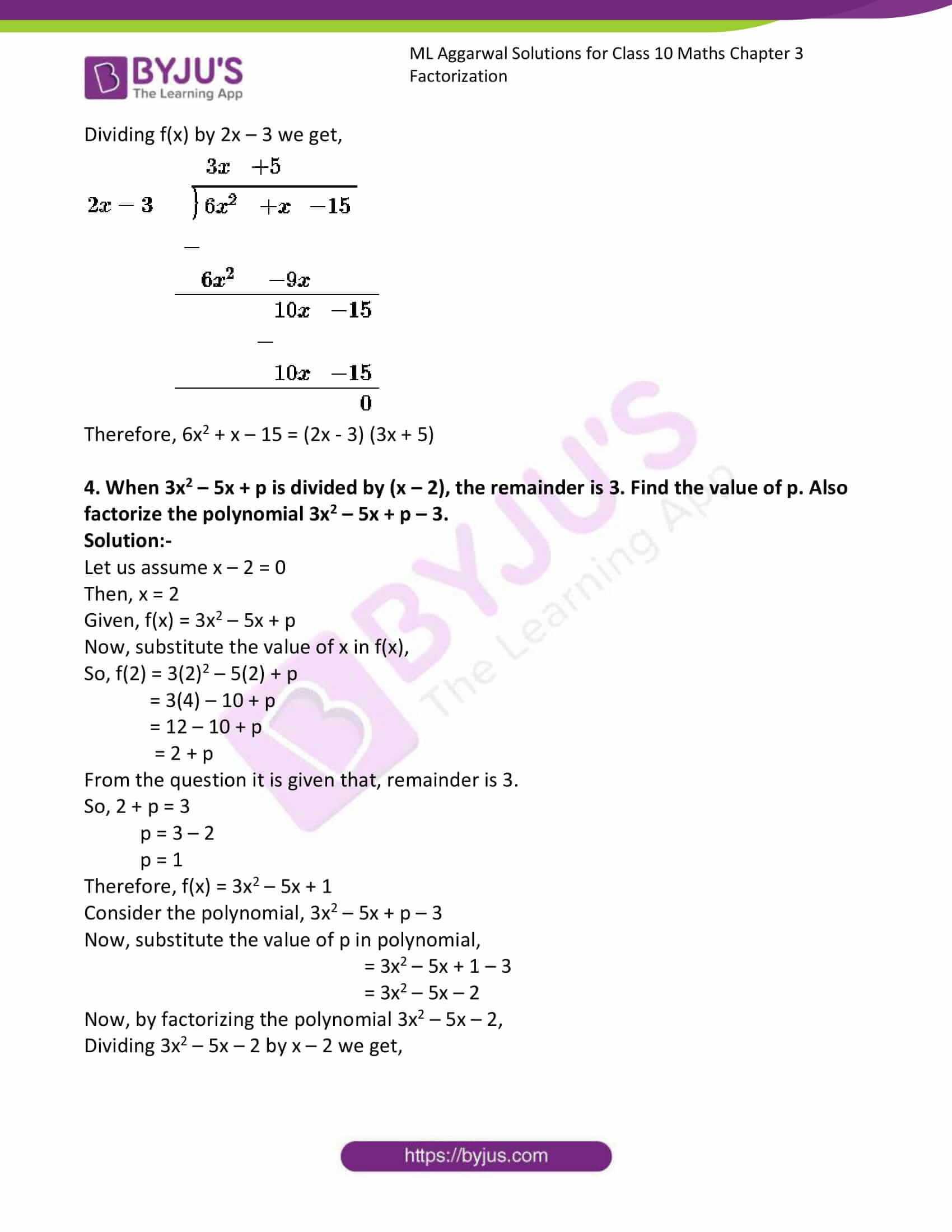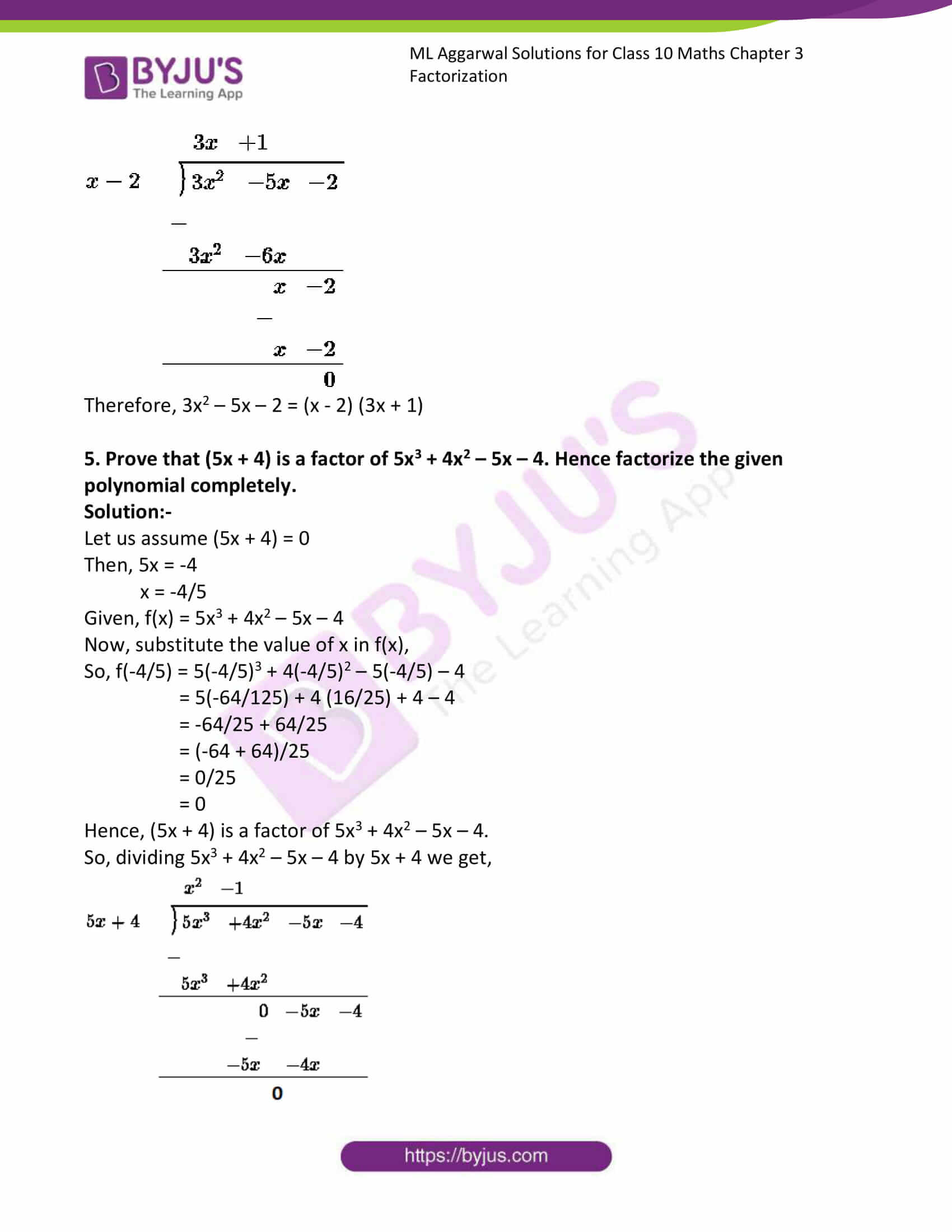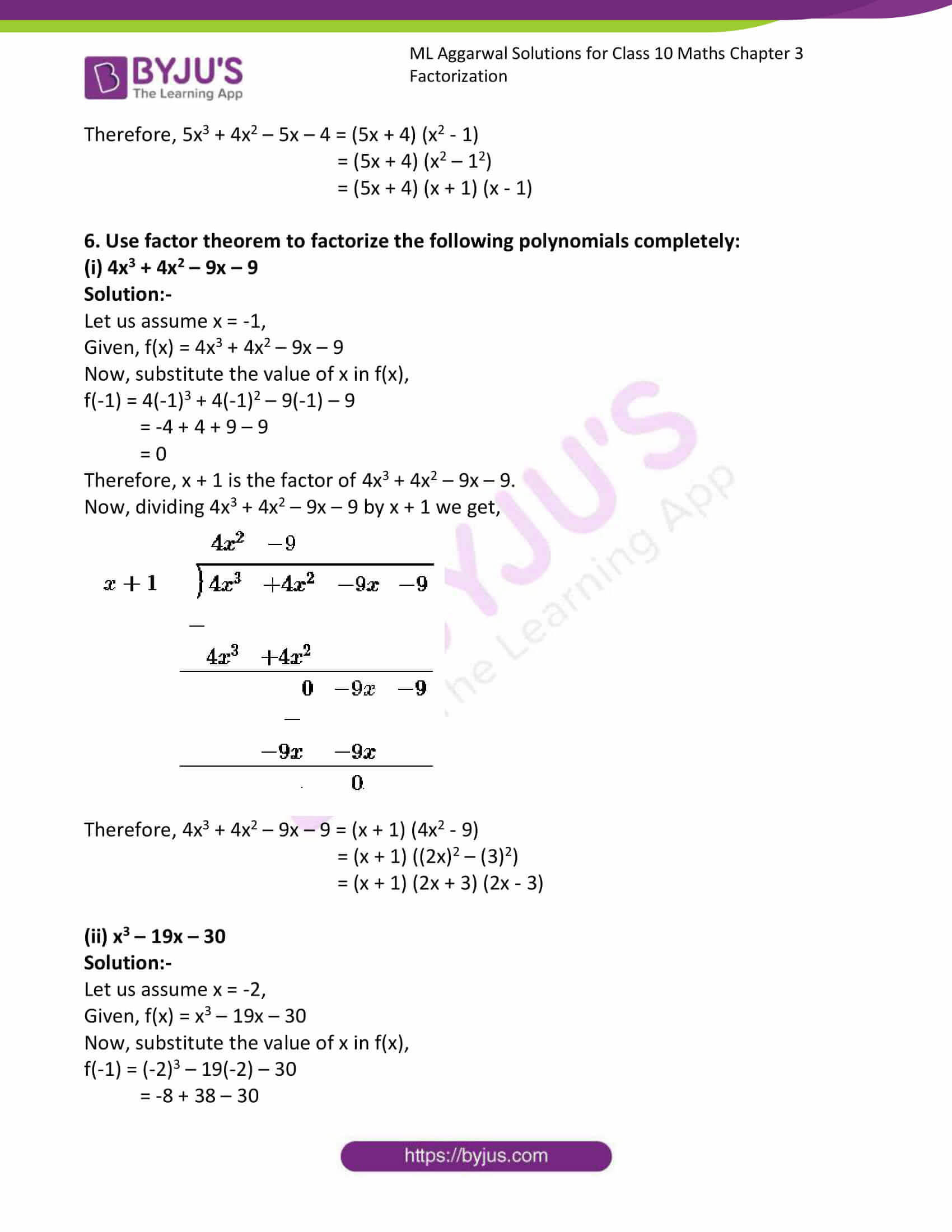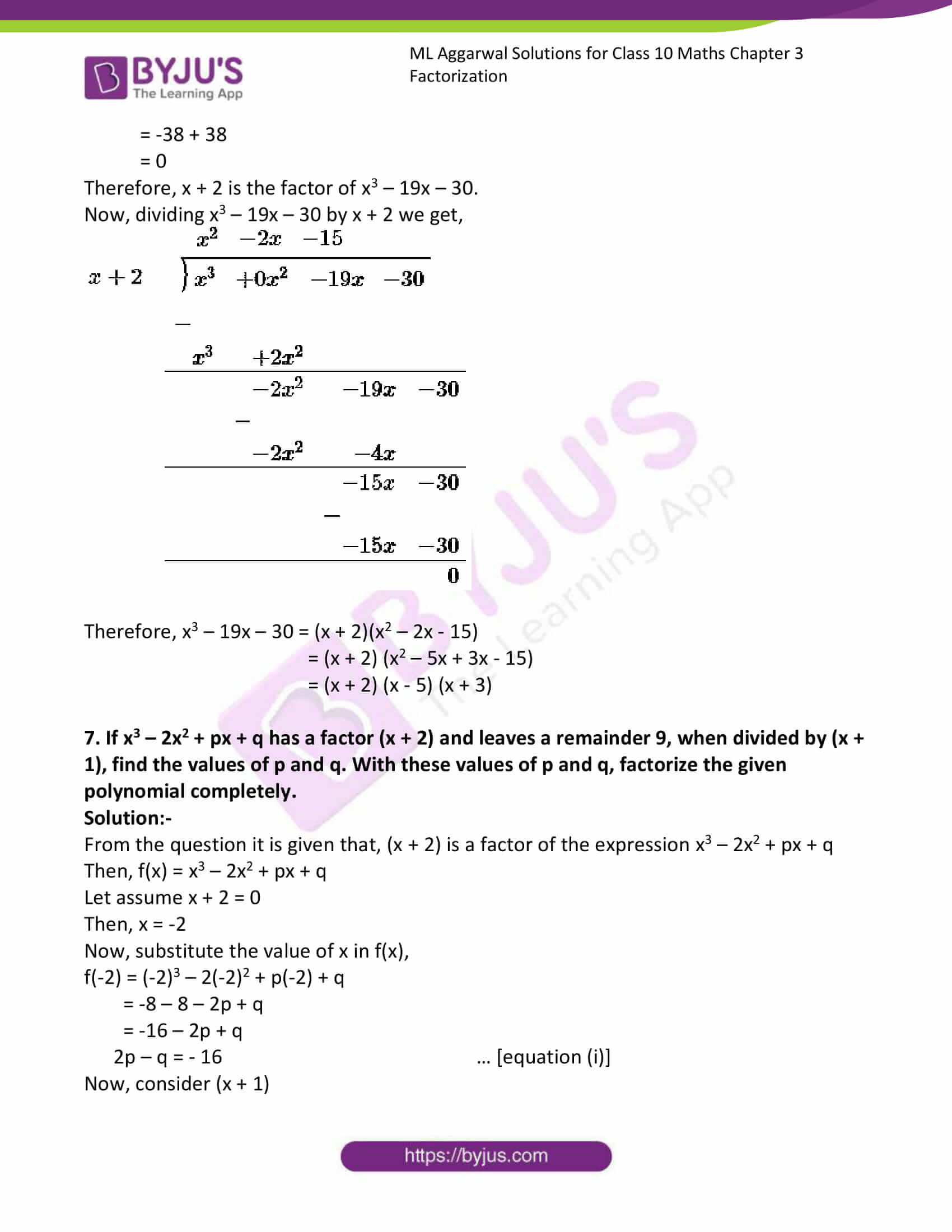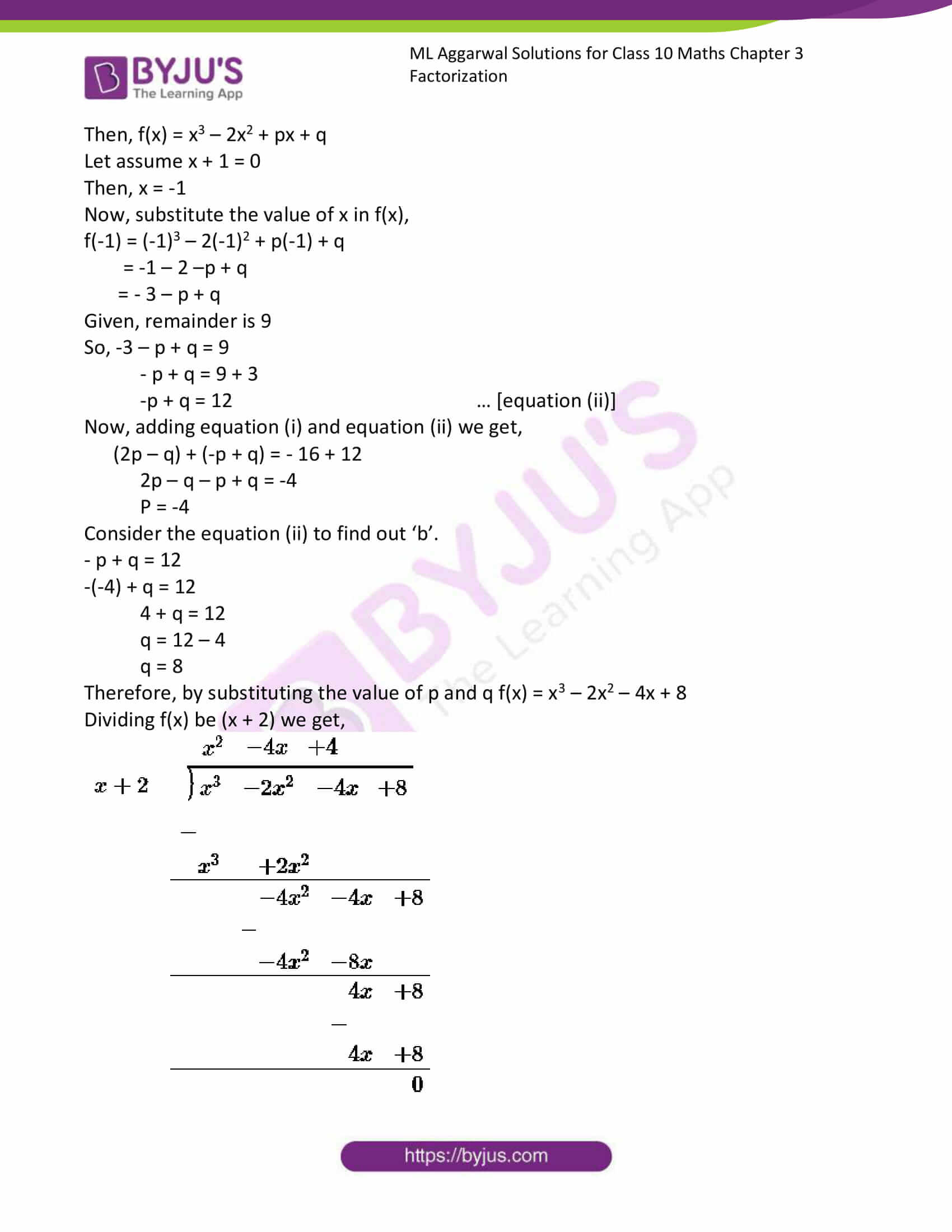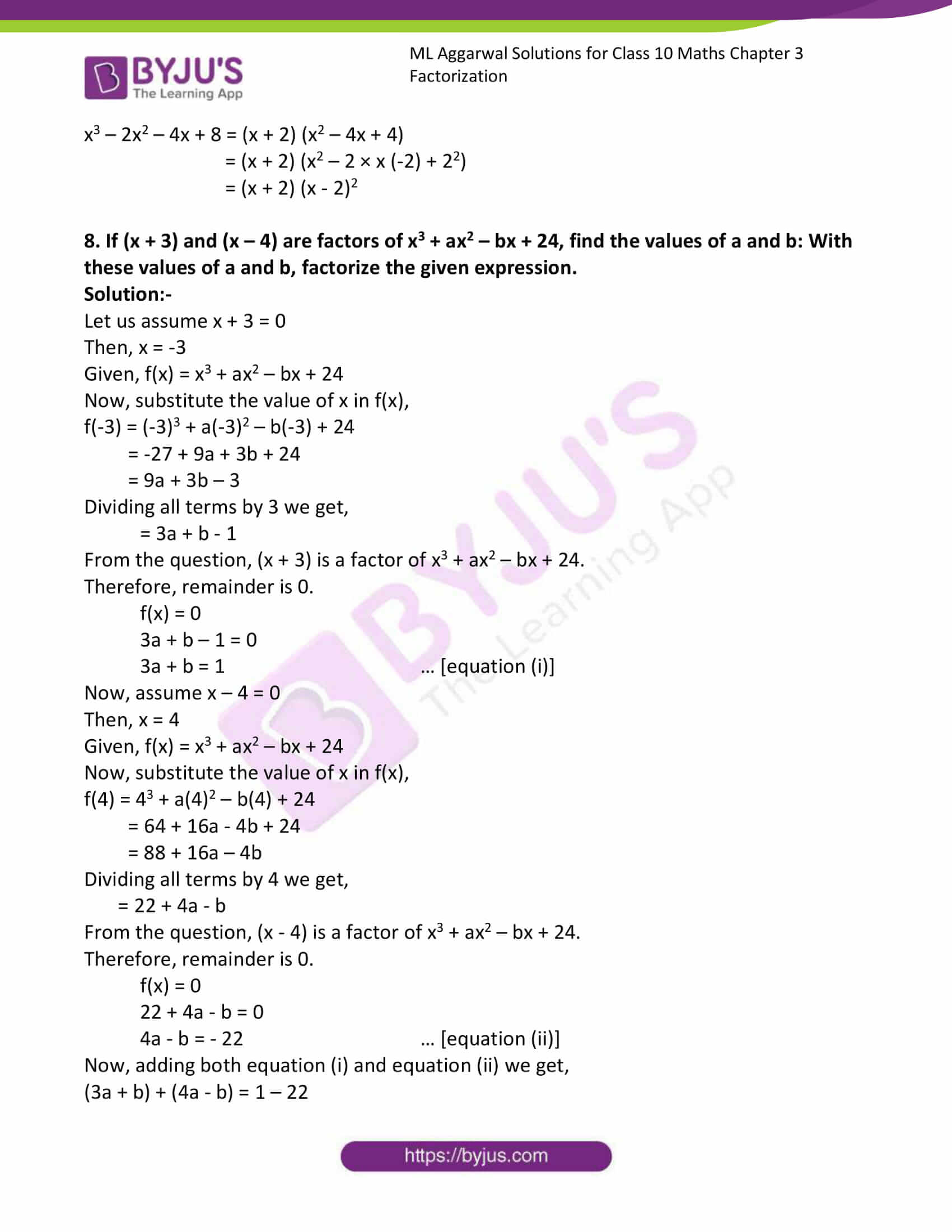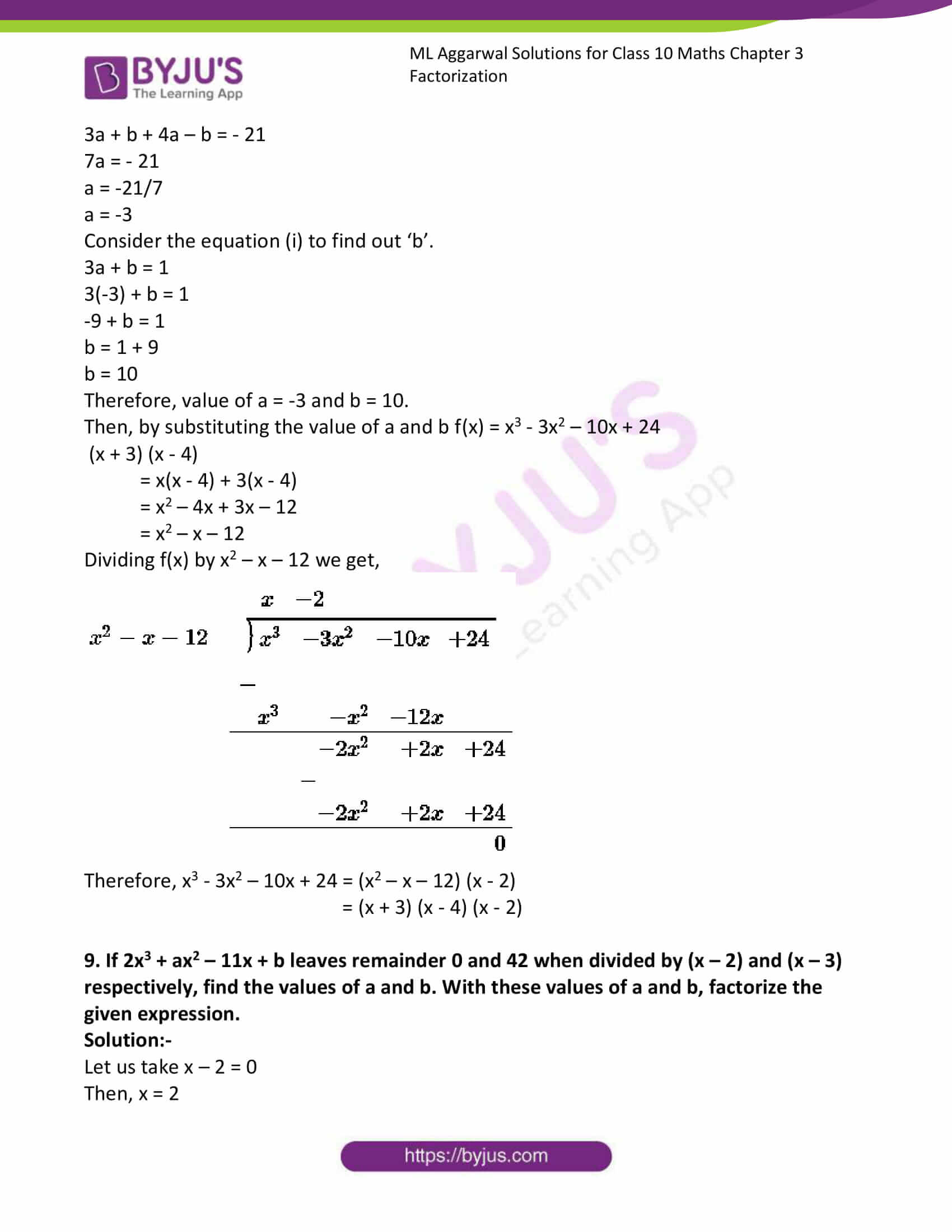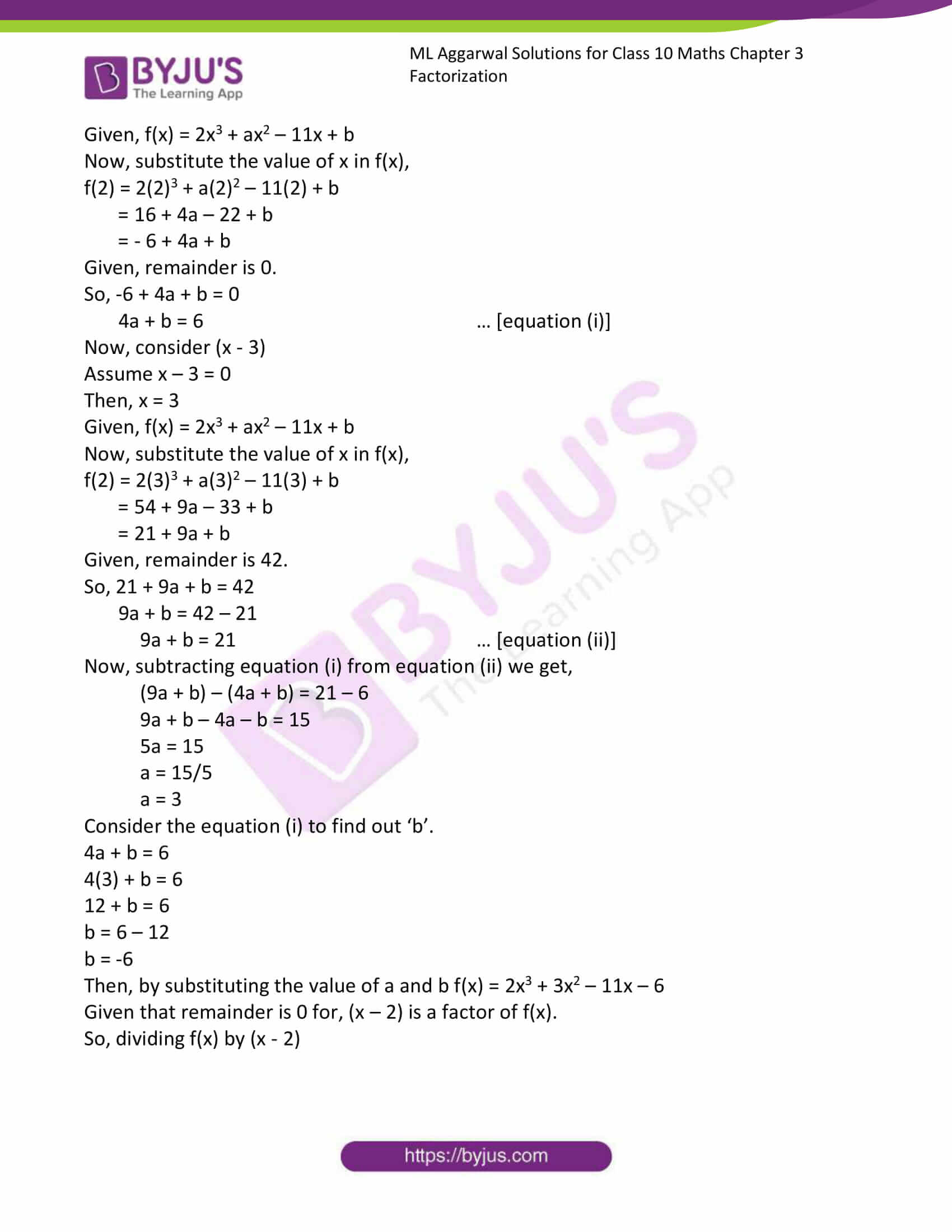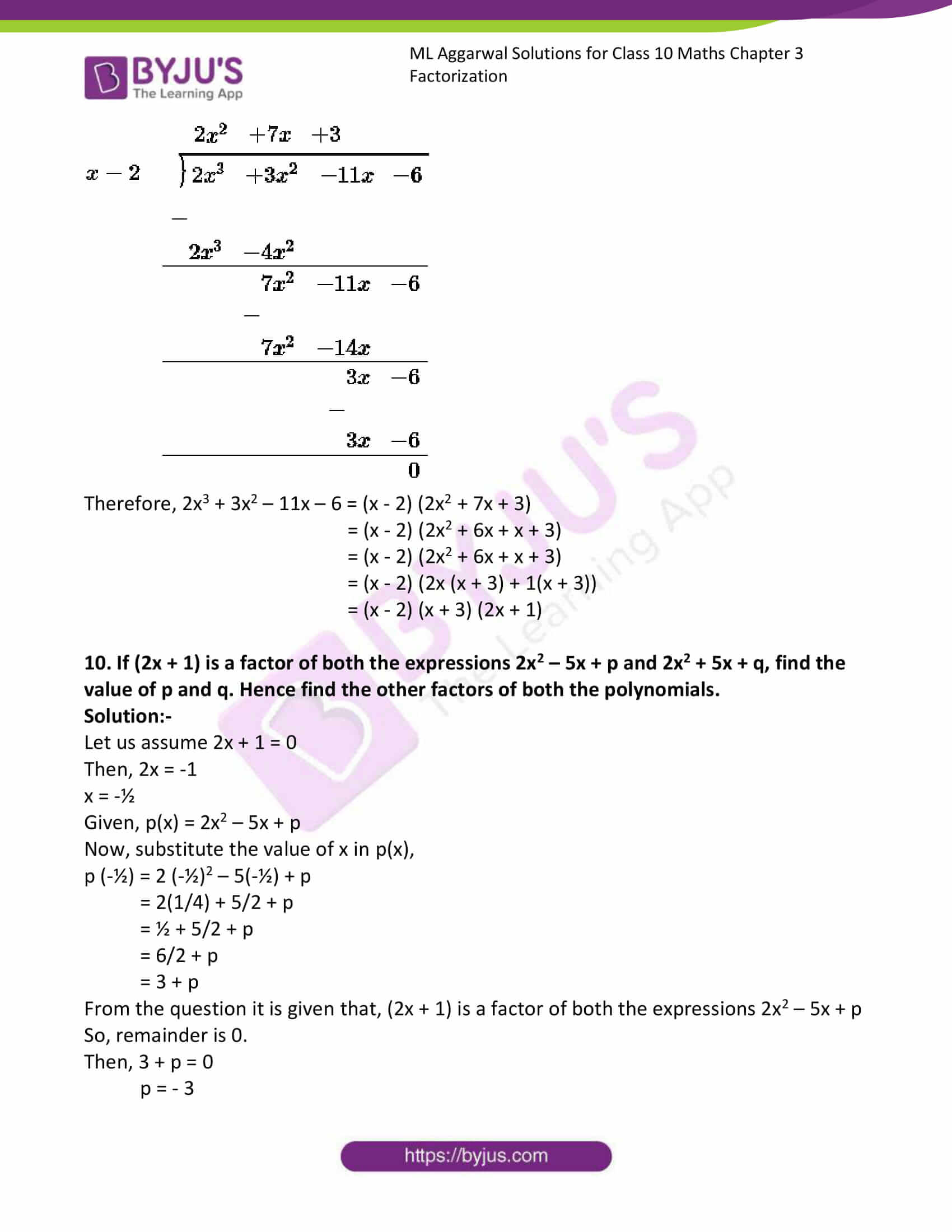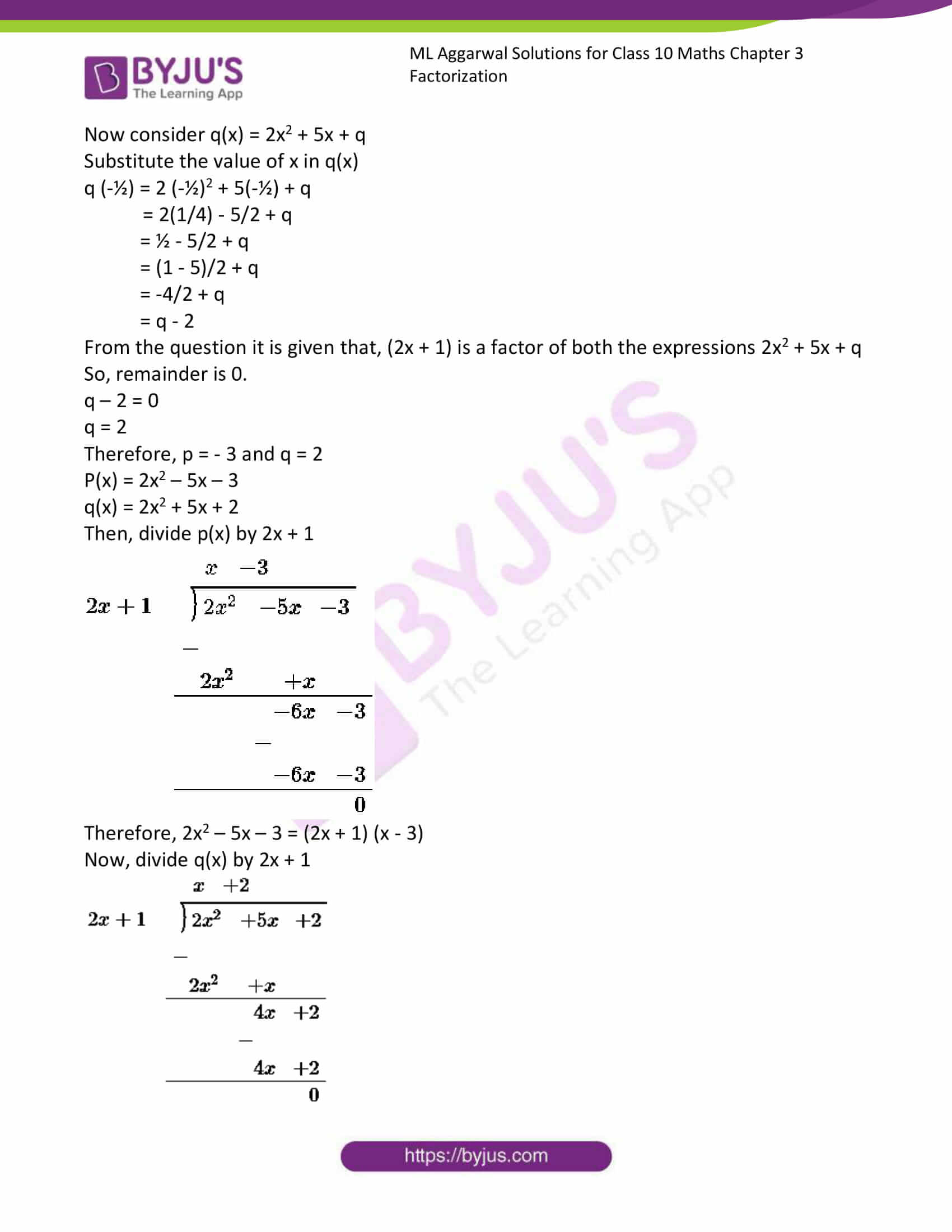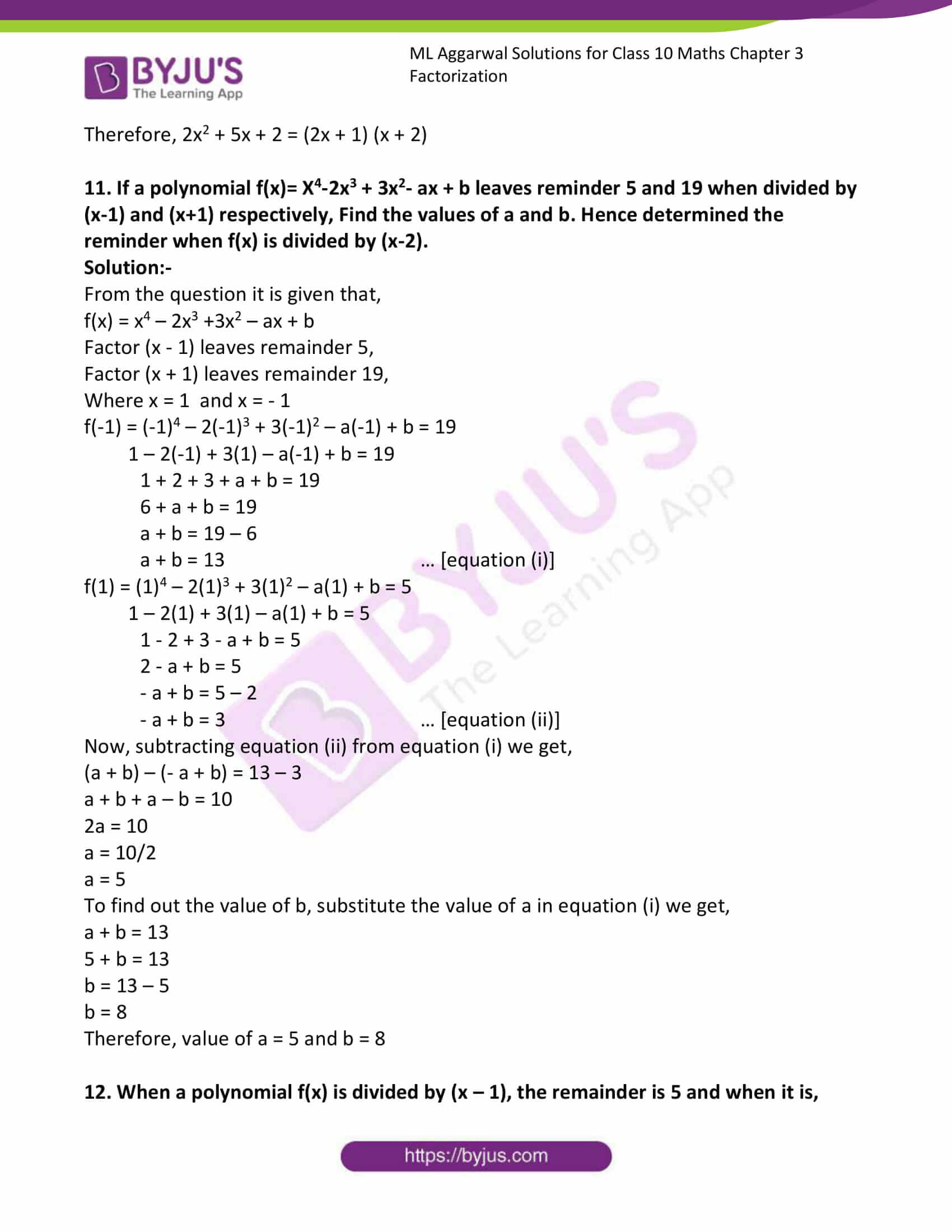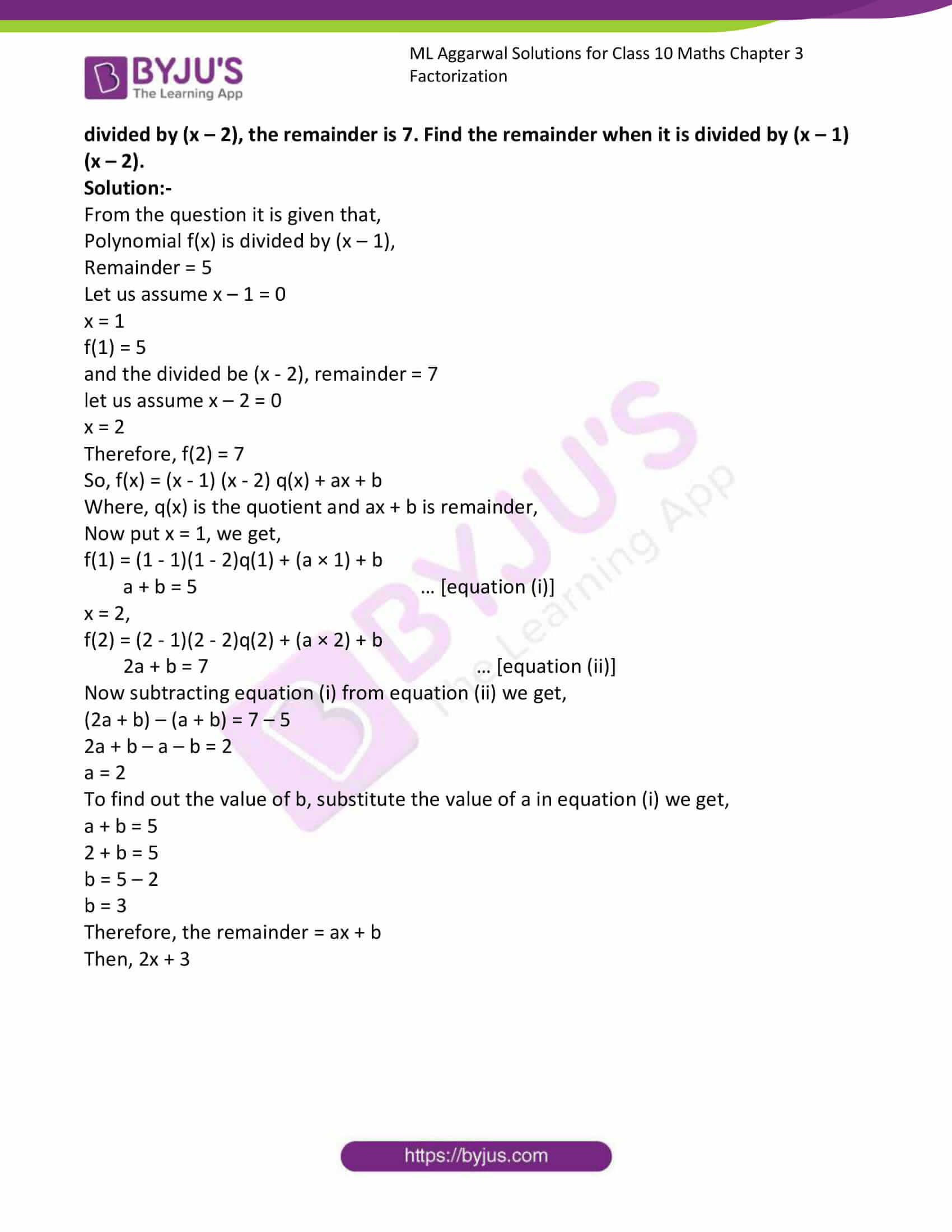### Access answers to ML Aggarwal Solutions for Class 10 Maths Chapter 6 Factorization

1. Find the remainder (without division) on dividing f(x) by (x – 2) where

(i) f(x) = 5x2 – 7x + 4

Solutions:-

Let us assume x – 2 = 0

Then, x = 2

Given, f(x) = 5x2 – 7x + 4

Now, substitute the value of x in f(x),

f(2)= (5 × 22) – (7 × 2) + 4

= (5 × 4) – 14 + 4

= 20 – 14 + 4

= 24 – 14

= 10

Therefore, the remainder is 10.

(ii) f(x) = 2x3 – 7x2 + 3

Solution:-

Let us assume x – 2 = 0

Then, x = 2

Given, f(x) = 2x3 – 7x2 + 3

Now, substitute the value of x in f(x),

f(2)= (2 × 23) – (7 × 22) + 3

= (2 × 8) – (7 × 4) + 3

= 16 – 28 + 3

= 19 – 28

= -9

Therefore, the remainder is -9.

2. Using the remainder theorem, find the remainder on dividing f(x) by (x + 3) where

(i) f(x) = 2x2 – 5x + 1

Solution:-

Let us assume x + 3 = 0

Then, x = -3

Given, f(x) = 2x2 – 5x + 1

Now, substitute the value of x in f(x),

f(-3)= (2 × -32) – (5 × (-3)) + 1

= (2 × 9) – (-15) + 1

= 18 + 15 + 1

= 34

Therefore, the remainder is 34.

(ii) f(x) = 3x3 + 7x2 – 5x + 1
Solution:-

Let us assume x + 3 = 0

Then, x = -3

Given, f(x) = 3x3 + 7x2 – 5x + 1

Now, substitute the value of x in f(x),

f(-3)= (3 × -33) + (7 × -32) – (5 × -3) + 1

= (3 × -27) + (7 × 9) – (-15) + 1

= – 81 + 63 + 15 + 1

= -81 + 79

= -2

Therefore, the remainder is -2.

3. Find the remainder (without division) on dividing f(x) by (2x + 1) where,

(i) f(x) = 4x2 + 5x + 3

Solution:-

Let us assume 2x + 1 = 0

Then, 2x = -1

X = -½

Given, f(x) = 4x2 + 5x + 3

Now, substitute the value of x in f(x),

f (-½) = 4 (-½)2 + 5 (-½) + 3

= (4 × ¼) + (-5/2) + 3

= 1 – 5/2 + 3

= 4 – 5/2

= (8 – 5)/2

= 3/2 = 1½

Therefore, the remainder is 1½.

(ii) f(x) = 3x3 – 7x2 + 4x + 11

Solution:-

Let us assume 2x + 1 = 0

Then, 2x = -1

X = -½

Given, f(x) = 3x3 – 7x2 + 4x + 11

Now, substitute the value of x in f(x),

f(-½) = (3 × (-½)3) – (7 × (-½)2 + (4 × -½) + 11

= 3 × (-1/8) – (7 × ¼) + (- 2) + 11

= -3/8 – 7/4 – 2 + 11

= – 3/8 – 7/4 + 9

= (-3 – 14 + 72)/8

= 55/8

=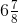4. (i) find the remainder (without division) when 2x3 – 3x2 + 7x – 8 is divided by x – 1.

Solution:-

Let us assume x – 1 = 0

Then, x = 1

Given, f(x) = 2x3 – 3x2 + 7x – 8

Now, substitute the value of x in f(x),

f(1) = (2 × 13) – (3 × 12) + (7 × 1) – 8

= 2 – 3 + 7 – 8

= 9 – 11

= – 2

(ii) Find the remainder (without division) on dividing 3x2 + 5x – 9 by (3x + 2).

Solution:-

Let us assume 3x + 2 = 0

3x = -2

x = -2/3

Then, x = -2/3

Given, f(x) = 3x2 + 5x – 9

Now, substitute the value of x in f(x),

f(-2/3) = (3 × (-2/3)2) + (5 × (-2/3)) – 9

= (3 × (4/9)) + (-10/3) – 9

= 4/3 – 10/3 – 9

= ((4 – 10)/3) – 9

= -6/3 – 9

= – 2 – 9

= -11

5. Using remainder theorem, find the value of k if on dividing 2x3 + 3x2 – kx + 5 by x – 2 leaves a remainder 7.

Solution:-

Let us assume, x – 2 = 0

Then, x = 2

Given, 2x3 + 3x2 – kx + 5

Now, substitute the value of x in f(x),

f(2) = (2 × 23) + (3 × 22) – (k × 2) + 5

= (2 × 8) + (3 × 4) – 2k + 5

= 16 + 12 – 2k + 5

= 33 – 2k

Form the question it is given that, remainder is 7.

So, 7 = 33 – 2k

2k = 33 – 7

2k = 26

K = 26/2

K = 13

Therefore, the value of k is 13.

6. Using remainder theorem, find the value of ‘a’ if the division of x3 + 5x2 – ax + 6 by (x – 1) leaves the remainder 2a.

Solution:-

Let us assume x -1 = 0

Then, x = 1

Given, f(x) = x3 + 5x2 – ax + 6

Now, substitute the value of x in f(x),

f(1) = 13 + (5 × 12) – (a × 1) + 6

= 1 + 5 – a + 6

= 12 – a

From the question it is given that, remainder is 2a

So, 2a = 12 – a

2a + a = 12

3a = 12

a = 12/3

a = 4

Therefore, the value of a is 4.

7.

(i) What number must be divided be subtracted from 2x2 – 5x so that the resulting polynomial leaves the remainder 2, when divided by 2x + 1?

Solution:-

let us assume ‘p’ be subtracted from 2x2 – 5x

So, dividing 2x2 – 5x by 2x + 1,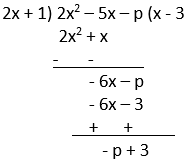Hence, remainder is 3 – p

From the question it is given that, remainder is 2.

3 – p = 2

p = 3 – 2

p = 1

Therefore, 1 is to be subtracted.

(ii) What number must be added to 2x3 – 7x2 + 2x so that the resulting polynomial leaves the remainder – 2 when divided by 2x – 3?

Solution:-

let us assume ‘p’ be subtracted from 2x3 – 7x2 + 2x,

So, dividing it by 2x – 3,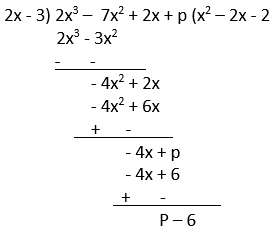Hence, remainder is p – 6

From the question it is given that, remainder is – 2.

P – 6 = – 2

P = -2 + 6

P = 4

Therefore, 4 is to be added.

8.

(i) When divided by x – 3 the polynomials x3 – px2 + x + 6 and 2x3 – x2 – (p + 3) x – 6 leave the same remainder. Find the value of ‘p’.

Solution:-

From the question it is given that, by dividing x3 – px2 + x + 6 and 2x3 – x2 – (p + 3)x – 6 by x – 3 = 0, then x = 3.

Let us assume p(x) = x3 – px2 + x + 6

Now, substitute the value of x in p(x),

p(3) = 33 – (p × 32) + 3 + 6

= 27 – 9p + 9

= 36 – 9p

Then, q(x) = 2x3 – x2 – (p + 3)x – 6

Now, substitute the value of x in q(x),

q(3) = (2 × 33) – (3)2 – (p + 3) × 3 – 6

= (2 × 27) – 9 – 3p – 9 – 6

= 54 – 24 – 3p

= 30 – 3p

Given, the remainder in each case is same,

So, 36 – 9p = 30 – 3p

36 – 30 = 9p – 3p

6 = 6p

p = 6/6

p = 1

Therefore, value of p is 1.

(ii) Find ‘a’ if the two polynomials ax3 + 3x2 – 9 and 2x3 + 4x + a, leaves the same remainder when divided by x + 3.

Solution:-

Let us assume p(x) = ax3 + 3x2 – 9 and q(x) =2x3 + 4x + a

From the question it is given that, both p(x) and q(x) leaves the same remainder when divided by x + 3.

Let us assume that, x + 3 = 0

Then, x = -3

Now, substitute the value of x in p(x) and in q(x),

So, p(-3) = q(-3)

a(-3)3 + 3(-3)2 – 9 = 2(-3)3 + 4(-3) + a

-27a + 27 – 9 = – 54 – 12 + a

-27a + 18 = – 66 + a

-27a – a = -66 – 18

-28 a = -84

a = 84/28

Therefore, a = 3

9. By factor theorem, show that (x + 3) and (2x – 1) are factors of 2x2 + 5x – 3.

Solution:-

Let us assume, x + 3 = 0

Then, x = – 3

Given, f(x) = 2x2 + 5x – 3

Now, substitute the value of x in f(x),

f(-3) = (2 × (-3)2) + (5 × -3) – 3

= (2 × 9) + (-15) – 3

= 18 – 15 – 3

= 18 – 18

= 0

Now, 2x – 1 = 0

Then, 2x = 1

x = ½

Given, f(x) = 2x2 + 5x – 3

Now, substitute the value of x in f(x),

f(½) = (2 × (½)2) + (5 × ½) – 3

= (2 × (¼)) + 5/2 – 3

= ½ + 5/2 – 3

= (1 + 5)/2 – 3

= 6/2 – 3

= 3 -3

= 0

Hence, it is proved that, (x + 3) and (2x – 1) are factors of 2x2 + 5x – 3.

10. Without actual division, prove that x4 + 2x3 – 2x2 + 2x + 3 is exactly divisible by x2 + 2x – 3.

Solution:-

Consider x2 + 2x – 3

By factor method, x2 + 3x – x – 3

= x (x + 3) – 1(x + 3)

= (x – 1) (x + 3)

So, f(x) = x4 + 2x3 – 2x2 + 2x + 3

Now take, x + 3 = 0

X = -3

Then, f(-3) = (-3)4 + 2 × -(33) – (2 × (-3)2) + (2 × -3) + 3

= 81 – 54 – 18 – 6 – 3

= 0

Therefore, (x + 3) is a factor of f(x)

And also, take x – 1 = 0

X = 1

Then, f(1) = 14 + 2(1)3 – 2(1)2 + 2(1) – 3

= 0

Therefore, (x – 1) is a factor of f(x)

By comparing both results, p(x) is exactly divisible by x2 + 2x – 3.

11. Show that (x – 2) is a factor of 3x2 – x – 10. Hence factories 3x2 – x – 10.

Solution:-

Let us assume x – 2 = 0

Then, x = 2

Given, f(x) = 3x2 – x – 10

Now, substitute the value of x in f(x),

f(2) = (3 × 22) – 2 – 10

= (3 × 4) – 2 – 10

= 12 – 2 – 10

= 12 – 12

= 0

Therefore, (x – 2) is a factor of f(x)

Then, dividing (3x2 – x – 10) by (x – 2), we get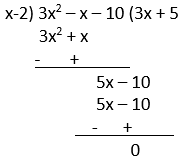Therefore, 3x2 – x – 10 = (x – 2) (3x + 5)

12.

(i) Show that, (x – 1) is a factor of x3 – 5x2 – x + 5 hence factories x3 – 5x2 – x + 5.

Solution:-

let us assume, x – 1 = 0

Then, x = 1

Given, f(x) = x3 – 5x2 – x + 5

Now, substitute the value of x in f(x),

f(1) = 13 – (5 × 12) – 1 + 5

= 1 – 5 – 1 + 5

= -6 + 6

= 0

Therefore, (x – 1) is a factor of x3 – 5x2 – x + 5

Then, dividing f(x) by (x – 1), we get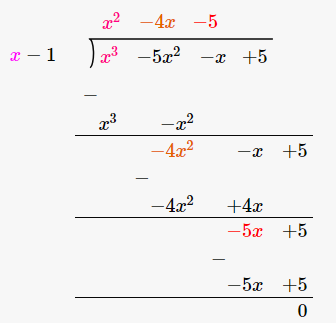Therefore, x3 – 5x2 – x + 5 = (x – 1) (x2 – 4x – 5)

= (x – 1) (x2 – 5x + x – 5)

= (x – 1) (x (x – 5) + 1 (x – 5))

= (x – 1) (x + 1) (x – 5)

(ii) Show that (x – 3) is a factor of x3 – 7x2 + 15x – 9. Hence factorize x3 – 7x2 + 15 x – 9.

Solution:-

let us assume, x – 3 = 0

Then, x = 3

Given, f(x) = x3 – 7x2 + 15x – 9

Now, substitute the value of x in f(x),

f(3) = 33 – (7 × 32) – (15 × 3) – 9

= 27 – (7 × 9) – 45 – 9

= 27 – 63 – 45 – 9

= 0

Therefore, (x – 3) is a factor of x3 – 7x2 + 15x – 9

Then, dividing f(x) by (x – 3), we get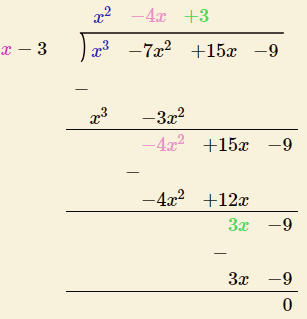Therefore, x3 – 7x2 + 15 – 9 = (x – 3) (x2 – 4x + 3)

= (x – 3) (x2 – x – 3x + 3)

= (x – 3) (x(x – 1) – 3(x – 1))

= (x – 3) (x – 3) (x – 1)

= (x – 3)2 (x – 1)

13. Show that (2x + 1) is a factor of 4x3 + 12x2 + 11x + 3. Hence factorize 4x3 + 12x2 + 11x + 3.

Solution:-

Let us assume 2x + 1 = 0

Then, 2x = -1

X = -½

Given, f(x) = 4x3 + 12x2 + 11x + 3

Now, substitute the value of x in f(x),

f(-½) = 4 (-½)3 + 12 (-½)2 + 11 (-½) + 3

= 4 (-1/8) + 12(1/4) + (-11/2) + 3

= -½ + 3 – 11/2 + 3

= (-1 – 11)/2 + 6

= -12/2 + 6

= -6 + 6

= 0

Therefore, (2x + 1) is a factor of 4x3 + 12x2 + 11x + 3

Then, dividing f(x) by (2x + 1), we get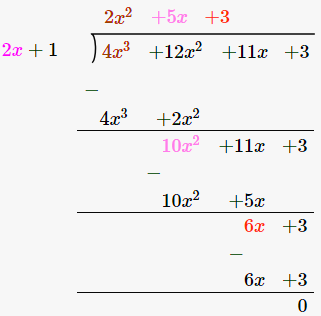Therefore, 4x3 + 12x2 + 11x + 3 = (2x + 1) (2x2 + 5x + 3)

= (2x + 1) (2x2 + 2x + 3x + 3)

= (2x + 1) (2x (x + 1) + 3(x + 1))

= (x + 1) (2x + 1) (2x + 3)

14. Show that 2x + 7 is a factor of 2x3 + 5x2 – 11x – 14. Hence factorize the given expression completely, using the factor theorem.

Solution:-

Let us assume 2x + 7 = 0

Then, 2x = -7

X = -7/2

Given, f(x) = 2x3 + 5x2 – 11x – 14

Now, substitute the value of x in f(x),

f(-7/2) = 2(-7/2)3 + 5(-7/2)2 + 11(-7/2) – 14

= 2(-343/8) + 5(49/4) + (-77/2) – 14

= -343/4 + 245/4 – 77/2 – 14

= (-343 + 245 + 154 – 56)/4

= -399 + 399/4

= 0

Therefore, (2x + 7) is a factor of 2x3 + 5x2 – 11x – 14

Then, dividing f(x) by (2x + 1), we get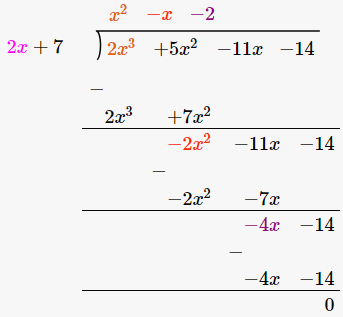Therefore, 2x3 + 5x2 – 11x – 14 = (2x + 7) (x2 – x – 2)

= (2x + 7) (x2 – 2x + x – 2)

= (2x + 7) (x(x – 2) + 1 (x – 2))

= (x + 1) (x – 2) (2x + 7)

15. Use factor theorem to factorize the following polynomials completely.

(i) x3 + 2x2 – 5x – 6

Solution:-

Let us assume x = -1,

Given, f(x) = x3 + 2x2 – 5x – 6

Now, substitute the value of x in f(x),

f(-1) = (-1)3 + 2(-1)2 – 5(-1) – 6

= -1 +2 (1) + 5 – 6

= -1 +2 + 5 – 6

= -7 + 7

= 0

Then, dividing f(x) by (x + 1), we get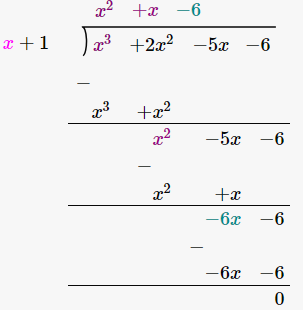Therefore, x3 + 2x2 – 5x – 6 = (x + 1) (x2 + 3x – 2x – 6)

= (x + 1) (x(x + 3) – 2(x + 3))

= (x + 1) (x – 2) (x + 3)

(ii) x3 – 13x – 12

Solution:-

Let us assume x = -1,

Given, f(x) = x3 – 13x – 12

Now, substitute the value of x in f(x),

f(-1) = (-1)3 – 13(-1) – 12

= -1 + 13 – 12

= – 13 + 13

= 0

Then, dividing f(x) by (x + 1), we get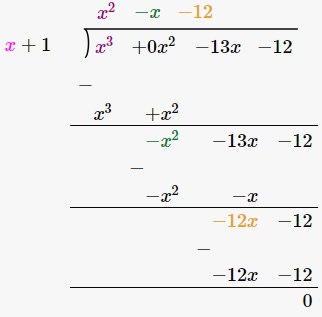Therefore, x3 – 13x – 12 = (x + 1) (x2 – x – 12)

= (x + 1) (x2 – 4x + 3x – 12)

= (x + 1) (x(x – 4)) + 3(x – 4))

= (x + 1) (x + 3) (x – 4)

16. Use the remainder theorem to factorize the following expression.

(i) 2x3 + x2 – 13x + 6

Solution:-

Let us assume x = 2,

Then, f(x) = 2x3 + x2 – 13x + 6

Now, substitute the value of x in f(x),

f(2) = (2 × 23) + 22 – 13 × 2 + 6

= (2 × 8) + 4 – 26 + 6

= 16 + 4 – 26 + 6

= 26 – 26

= 0

Then, dividing f(x) by (x – 2), we get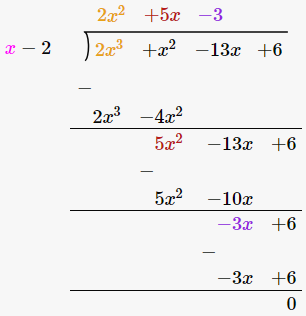Therefore, 2x3 + x2 – 13x + 6 = (x – 2) (2x2 + 5x – 3)

= (x – 2)(2x2 + 6x – x – 3)

= (x – 2) (2x(x + 3) – 1 (x + 3))

= (x – 2) (x + 3) (2x – 1)

(ii) 3x2 + 2x2 – 19x + 6

Solution:-

Given, f(x) = 3x3 + 2x2 – 19x + 6

Let us assume x = 1

Then, f(1) = 3(1)3 + 2(1)2 – (19 × 1) + 6

= 3 + 2 – 19 + 6

= 11 – 19

= – 8

So, – 8 ≠ 0

Let us assume x = -1

Then, f(-1) = 3(-1)3 + 2(-1)2 – (19 × (-1)) + 6

= – 3 + 2 + 19 + 6

= – 3 + 27

= 24

So, 24 ≠ 0

Now, assume x = 2

Then, f(2) = 3(2)3 + 2(2)2 – (19 × (2)) + 6

= 24 + 8 – 38 + 6

= 38 – 38

= 0

So, 0 = 0

Therefore, (x – 2) is a factor of f(x).

f(x) = 3x3 + 2x2 – 19x + 6

= 3x3 – 6x2 + 8x2 – 16x – 3x + 6

= 3x2 (x – 2) + 8x (x – 2) – 3(x – 2)

= (x – 2) (3x2 + 8x – 3)

= (x – 2) (3x2 + 9x – x – 3)

= (x – 2) (3x(x + 3) – 1(x + 3)

= (x – 2) (x + 3) (3x – 1)

(iii) 2x3 + 3x2 – 9x – 10

Solution:-

Given, f(x) = 2x3 + 3x2 – 9x – 10

Let us assume, x = -1

= 2(-1)3 + 3(-1)2 – 9 (-1) – 10

= -2 + 3 + 9 – 10

= 12 – 12

= 0

Therefore, (x + 1) is the factor of 2x3 + 3x2 – 9x – 10

Then, dividing f(x) by (x + 1), we get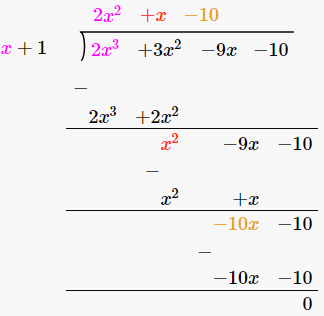Therefore, 2x3 + 3x2 – 9x – 10 = 2x2 + 5x – 4x – 10

= x(2x + 5) – 2 (2x + 5) – (2x + 5) (x – 2)

Hence the factors are (x + 1) (x – 2) (2x + 5)

17. using the remainder and factor theorem factorize the following polynomial

x3 + 10x2 – 37x + 26

Solution:-

Given, f(x) = x3 + 10x2 – 37x + 26

Let us assume, x = 1

Then, f(1) = 13 + 10(1)2 – 37 (1) + 26

= 1 + 10 – 37 + 26

= 37 – 37

= 0

Therefore, x – 1is a factor of x3 + 10x2 – 37x + 26

Then, dividing f(x) by (x – 1), we get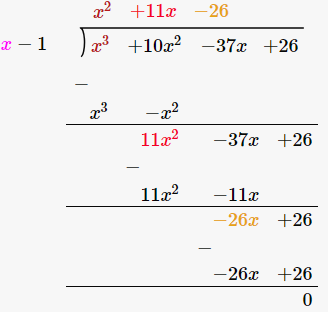Therefore, x3 + 10x2 – 37x + 26 = (x – 1) (x2 + 11x – 26)

= (x – 1) (x2 + 13x – 2x – 26)

= (x – 1) (x (x + 13) – 2(x + 13))

= (x – 1) ((x – 2) (x + 13))

18. If (2x + 1) is a factor of 6x3 + 5x2 + ax – 2 find the value of a.

Solution:-

Let us assume 2x + 1 = 0

Then, 2x = – 1

X = -½

Given, f(x) = 6x3 + 5x2 + ax – 2

Now, substitute the value of x in f(x),

f (-½) = 6 (-½)3 + 5 (-½)2 + a (-½) – 2

= 6 (-1/8) + 5 (¼) – ½a – 2

= -3/4 + 5/4 – a/2 – 2

= (-3 + 4 – 2a – 8)/4

= (-6 – 2a)/4

From the question, (2x + 1) is a factor of 6x3 + 5x2 + ax – 2

Then, remainder is 0.

So, (-6 – 2a)/4 = 0

-6 – 2a = 4 × 0

– 6 – 2a = 0

-2a = 6

a = -6/2

a = – 3

Therefore, the value of a is – 3.

19. If (3x – 2) is a factor of 3x3 – kx2 + 21x – 10, find the value of k.

Solution:-

Let us assume 3x – 2 = 0

Then, 3x = 2

X = 2/3

Given, f(x) = 3x3 – kx2 + 21x – 10

Now, substitute the value of x in f(x),

f (2/3) = 3 (2/3)3 – k (2/3)2 + 21 (2/3) – 10

= 3 (8/27) – k (4/9) + 14 – 10

= 8/9 – 4k/9 + 14 – 10

= 8/9 – 4k/9 + 4

= (8 – 4k + 36)/9

= (44 – 4k)/9

From the question, (3x – 2) is a factor of 3x3 – kx2 + 21x – 10

Then, remainder is 0

So, (44 – 4k)/9 = 0

44 – 4k = 0 × 9

44 = 4k

K = 44/4

K = 11

20. If (x – 2) is a factor of 2x3 – x2 + px – 2, then (i) find the value of p. (ii) with this value of p, factorize the above expression completely.

Solution:-

Let us assume x -2 = 0

Then, x = 2

Given, f(x) = 2x3 – x2 + px – 2

Now, substitute the value of x in f(x),

f(2) = (2 × 23) – 22 + (p × 2) – 2

= (2 × 8) – 4 + 2p – 2

= 16 – 4 + 2p – 2

= 16 – 6 + 2p

= 10 + 2p

From the question, (x – 2) is a factor of 2x3 – x2 + px – 2

Then, remainder is 0.

10 + 2p = 0

2p = – 10

P = -10/2

P = -5

So, (x – 2) is a factor of 2x3 – x2 + 5x – 2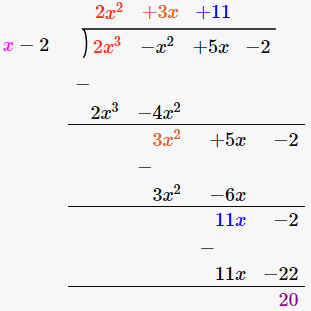Therefore, 2x3 – x2 + 5x – 2 = (x – 2) (2x2 + 3x + 1)

= (x – 2) (2x2 + 2x + x + 1)

= (x – 2) (2x(x + 1) + 1(x + 1))

= (x + 1) (x – 2) (2x + 1)

21. Find the value of ‘K’ for which x = 3 is a solution of the quadratic equation, (K + 2)x2 – Kx + 6 = 0. Also, find the other root of the equation.

Solution:-

From the question it is given that, x = 3

And (K + 2)x2 – Kx + 6 = 0

Kx2 + 2x2 – kx + 6 = 0

Now substitute the value of x,

K (3)2 + 2 (3)2 – k (3) + 6 = 0

9k + 18 – 3k + 6 = 0

6k + 24 = 0

K = -24/6

K = -4

Then, Kx2 + 2x2 – kx + 6 = 0

(-4)x2 + 2x2 – (-4)x + 6 = 0

-4x2 + 2x2 + 4x + 6 = 0

-2x2 + 4x + 6 = 0

Divide both the side by -2 we get,

X2 – 2x – 3 = 0

X2 – 3x + x – 3 = 0

X(x – 3) + 1(x – 3) = 0

(x – 3) (x + 1) = 0

(x – 3) = 0

X = 3

(x + 1) = 0

x = -1

Therefore, the other roots are x = – 1

22. What number should be subtracted from 2x3 – 5x2 + 5x so that the resulting polynomial has 2x – 3 as a factor?

Solution:-

Let us assume the number to be subtracted from 2x3 – 5x2 + 5x be p.

Then, f(x) = 2x3 – 5x2 + 5x – p

Given, 2x – 3 = 0

x = 3/2

f(3/2) = 0

So, f(3/2) = 2(3/2)3 – 5(3/2)2 + 5(3/2) – p = 0

2(27/8) – 5(9/4) + 15/2 – p = 0

27/4 – 45/4 + 15/2 – p = 0 [multiply by 4 for all numerator]

27 – 45 + 30 – 4p = 0

57 – 45 – 4p = 0

12 – 4p = 0

P = 12/4

P = 3

Therefore, 3 is the number should be subtracted from 2x3 – 5x2 + 5x.

23.

(i) Find the value of the constants a and b, if (x – 2) and (x + 3) are both factors of the expression x3 + ax2 + bx – 12.

Solution:-

Let us assume x – 2 = 0

Then, x = 2

Given, f(x) = x3 + ax2 + bx – 12

Now, substitute the value of x in f(x),

f(2) = 23 + a(2)2 + b(2) – 12

= 8 + 4a + 2b – 12

= 4a + 2b – 4

From the question, (x – 2) is a factor of x3 + ax2 + bx – 12.

So, 4a + 2b – 4 = 0

4a + 2b = 4

By dividing both the side by 2 we get,

2a + b = 2 … [equation (i)]

Now, assume x + 3 = 0

Then, x = -3

Given, f(x) = x3 + ax2 + bx – 12

Now, substitute the value of x in f(x),

f(-3) = (-3)3 + a(-3)2 + b(-3) – 12

= -27 + 9a – 3b – 12

= 9a – 3b – 39

From the question, (x – 3) is a factor of x3 + ax2 + bx – 12.

So, 9a – 3b – 39 = 0

9a – 3b = 39

By dividing both the side by 3 we get,

3a – b = 13 … [equation (ii)]

Now, adding both equation (i) and equation (ii) we get,

(2a + b) + (3a – b) = 2 + 13

2a + 3a + b – b = 15

5a = 15

a = 15/5

a = 3

Consider the equation (i) to find out ‘b’.

2a + b = 2

2(3) + b = 2

6 + b = 2

b = 2 – 6

b = -4

(ii) If (x + 2) and (x + 3) are factors of x3 + ax + b, Find the values of a and b.

Solution:-

Let us assume x + 2 = 0

Then, x = -2

Given, f(x) = x3 + ax + b

Now, substitute the value of x in f(x),

f(-2) = (-2)3 + a(-2) + b

= -8 – 2a + b

From the question, (x + 2) is a factor of x3 + ax + b.

Therefore, remainder is 0.

f(x) = 0

– 8 – 2a + b = 0

2a – b = – 8 … [equation (i)]

Let us assume x + 3 = 0

Then, x = -3

Given, f(x) = x3 + ax + b

Now, substitute the value of x in f(x),

f(-2) = (-3)3 + a(-3) + b

= -27 – 3a + b

From the question, (x + 3) is a factor of x3 + ax + b.

Therefore, remainder is 0.

f(x) = 0

– 27 – 3a + b = 0

3a – b = – 27 … [equation (i)]

Now, subtracting both equation (i) and equation (ii) we get,

(2a – b) – (3a – b) = -8 – (-27)

2a – 3a – b + b = – 8 + 27

-a = 19

a = -19

Consider the equation (i) to find out ‘b’.

2a – b = – 8

2(-19) – b = -8

-38 – b = – 8

b = -38 +8

b = -30

24. If (x + 2) and (x – 3) are factors of x3 + ax + b, find the values of a and b. With these values of a and b, factorize the given expression.

Solution:-

Let us assume x + 2 = 0

Then, x = -2

Given, f(x) = x3 + ax + b

Now, substitute the value of x in f(x),

f(-2) = (-2)3 + a(-2) + b

= -8 – 2a + b

From the question, (x + 2) is a factor of x3 + ax + b.

Therefore, remainder is 0.

f(x) = 0

– 8 – 2a + b = 0

2a – b = – 8 … [equation (i)]

Now, assume x – 3 = 0

Then, x = 3

Given, f(x) = x3 + ax + b

Now, substitute the value of x in f(x),

f(3) = (3)3 + a(3) + b

= 27 + 3a + b

From the question, (x – 3) is a factor of x3 + ax + b.

Therefore, remainder is 0.

f(x) = 0

27 + 3a + b = 0

3a + b = – 27 … [equation (ii)]

Now, adding both equation (i) and equation (ii) we get,

(2a – b) + (3a + b) = – 8 – 27

2a – b + 3a + b = -35

5a = -35

a = -35/5

a = -7

Consider the equation (i) to find out ‘b’.

2a – b = – 8

2(-7) – b = -8

-14 – b = -8

b = – 14 + 8

b = -6

Therefore, value of a = -7 and b = -6.

Then, f(x) = x3 – 7x – 6

(x + 2) (x – 3)

= x(x – 3) + 2(x – 3)

= x2 – 3x + 2x – 6

= x2 – x – 6

Dividing f(x) by x2 – x – 6 we get,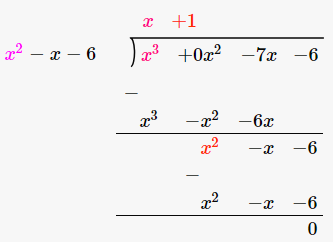Therefore, x3 – 7x – 6 = (x + 1) (x + 2) (x – 3)

25. (x – 2) is a factor of the expression x3 + ax2 + bx + 6. When this expression is divided by (x – 3), it leaves the remainder 3. Find the values of a and b.

Solution:-

From the question it is given that, (x – 2) is a factor of the expression x3 + ax2 + bx + 6

Then, f(x) = x3 + ax2 + bx + 6 … [equation (i)]

Let assume x – 2 = 0

Then, x = 2

Now, substitute the value of x in f(x),

f(2) = 23 + a(2)2 + 2b + 6

= 8 + 4a + 2b + 6

= 14 + 4a + 2b

By dividing the numbers by 2 we get,

= 7 + 2a + b

From the question, (x – 2) is a factor of the expression x3 + ax2 + bx + 6.

So, remainder is 0.

f(x) = 0

7 + 2a + b = 0

2a + b = -7 … [equation (ii)]

Now, expression is divided by (x – 3), it leaves the remainder 3.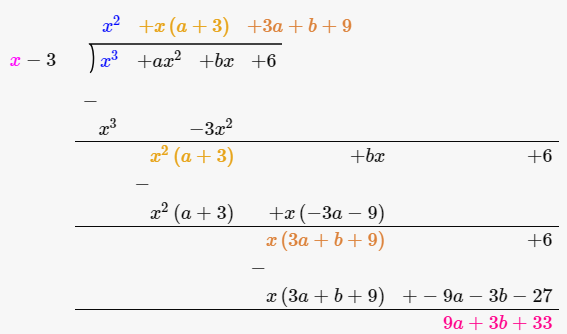So, remainder = 33 + 9a + 3b = 3

9a + 3b = 3 – 33

9a + 3b = -30

By dividing the numbers by 3 we get,

= 3a + b = – 10 … [equation (iii)]

Now, subtracting equation (iii) from equation (ii) we get,

(3a + b) – (2a + b) = – 10 – (-7)

3a – 2a + b – b = – 10 + 7

a = -3

Consider the equation (ii) to find out ‘b’.

2a + b = – 7

2(-3) + b = – 7

-6 + b = – 7

b = – 7 + 6

b = – 1

26. If (x – 2) is a factor of the expression 2x3 + ax2 + bx – 14 and when the expression is divided by (x – 3), it leaves a remainder 52, find the values of a and b.

Solution:-

From the question it is given that, (x – 2) is a factor of the expression 2x3 + ax2 + bx – 14

Then, f(x) = 2x3 + ax2 + bx – 14 … [equation (i)]

Let assume x – 2 = 0

Then, x = 2

Now, substitute the value of x in f(x),

f(2) = 2(2)3 + a(2)2 + 2b – 14

= 16 + 4a + 2b – 14

= 2 + 4a + 2b

By dividing the numbers by 2 we get,

= 1 + 2a + b

From the question, (x – 2) is a factor of the expression 2x3 + ax2 + bx – 14.

So, remainder is 0.

f(x) = 0

1 + 2a + b = 0

2a + b = -1 … [equation (ii)]

Now, expression is divided by (x – 3), it leaves the remainder 52.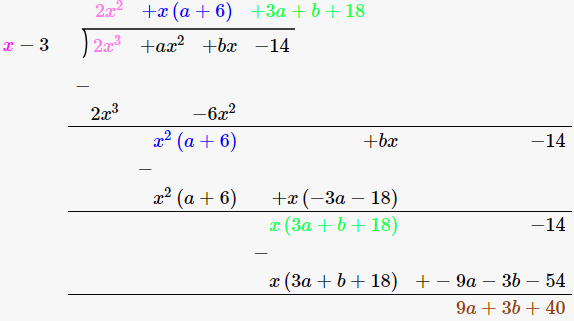So, remainder = 9a + 3b + 40 = 52

9a + 3b = 52 – 40

9a + 3b = 12

By dividing the numbers by 3 we get,

= 3a + b = 4 … [equation (iii)]

Now, subtracting equation (iii) from equation (ii) we get,

(3a + b) – (2a + b) = 4 – (-1)

3a – 2a + b – b = 4 + 1

a = 5

a = 5

Consider the equation (ii) to find out ‘b’.

2a + b = – 1

2(5) + b = – 1

10 + b = – 1

b = – 1 – 10

b = – 11

27. If ax3 + 3x2 + bx – 3 has a factor (2x + 3) and leaves remainder – 3 when divided by (x + 2), find the values of a and b. With these values of a and b, factorize the given expression.

Solution:-

Let us assume, 2x + 3 = 0

Then, 2x = -3

x = -3/2

Given, f(x) = ax3 + 3x2 + bx – 3

Now, substitute the value of x in f(x),

f(-3/2) = a(-3/2)3 + 3(-3/2)2 + b(-3/2) – 3

= a(-27/8) + 3(9/4) – 3b/2 – 3

= -27a/8 + 27/4 – 3b/2 – 3

From the question it is given that, ax3 + 3x2 + bx – 3 has a factor (2x + 3).

So, remainder is 0.

-27a/8 + 27/4 – 3b/2 – 3 = 0

-27a + 54 – 12b – 24 = 0

-27a – 12b = -30

By dividing the numbers by – 3 we get,

9a + 4b = 10 [equation (i)]

Now, let us assume x + 2 = 0

Then, x = -2

Given, f(x) = ax3 + 3x2 + bx – 3

Now, substitute the value of x in f(x),

f(2) = a(-2)3 + 3(-2)2 + b(-2) – 3

= -8a + 12 – 2b – 3

= -8a – 2b + 9

Leaves the remainder -3

So, -8a – 2b + 9 = -3

-8a – 2b = -3 – 9

-8a – 2b = -12

By dividing both sides by -2 we get,

4a + b = 6 [equation (ii)]

By multiplying equation (ii) by 4,

16a + 4b = 24

Now, subtracting equation (ii) from equation (i) we get,

(16a + 4b) – (9a + 4b) = 24 – 10

16a – 9a + 4b – 4b = 14

7a = 14

a = 14/7

a = 2

Consider the equation (i) to find out ‘b’.

9a + 4b = 10

9(2) + 4b = 10

18 + 4b = 10

4b = 10 – 18

4b = -8

b = -8/4

b = -2

Therefore, f(x) = ax3 + 3x2 + bx – 3

= 2x3 + 3x2 – 2x – 3

Given, 2x + 3 is a factor of f(x)

So, divide f(x) by 2x + 3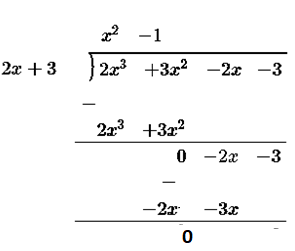Therefore, 2x3 + 3x2 – 2x – 3 = (2x + 3) (x2 – 1)

= (2x + 3) (x + 1) (x – 1)

28. Given f(x) = ax2 + bx + 2 and g(x) = bx2 + ax + 1. If x – 2 is a factor of f(x) but leaves the remainder – 15 when it divides g(x), find the values of a and b. With these values of a and b, factorise the expression. f(x) + g(x) + 4x2 + 7x.

Solution:-

From the question it is given that, f(x) = ax2 + bx + 2 and g(x) = bx2 + ax + 1 and x – 2 is a factor of f(x),

So, x = 2

Now, substitute the value of x in f(x),

f(2) = 0

a(2)2 + b(2) + 2 = 0

4a + 2b + 2 = 0

By dividing both sides by 2 we get,

2a + b + 1 = 0 … [equation (i)]

Given, g(x) divide by (x – 2), leaves remainder – 15

g(x) = bx2 + ax + 1

So, g(2) = -15

b(2)2 + 2a + 1 = -15

4b + 2a + 1 + 15 = 0

4b + 2a + 16 = 0

By dividing both sides by 2 we get,

2b + a + 8 = 0 … [equation (ii)]

Now, subtracting equation (ii) from equation (i) multiplied by 2,

(4a + 2b + 2) – (a + 2b + 8) = 0 – 0

4a – a + 2b – 2b + 2 – 8 = 0

3a – 6 = 0

3a = 6

a = 6/3

a = 2

Consider the equation (i) to find out ‘b’.

2a + b + 1 = 0

2(2) + b = – 1

4 + b = – 1

b = – 1 – 4

b = – 5

Now, f(x) = ax2 + bx + 2 = 2x2 – 5x + 2

g(x) = bx2 + ax + 1 = -5x2 + 2x + 1

then, f(x) + g(x) + 4x2 + 7x

= 2x2 – 5x + 2 – 5x2 + 2x + 1 + 4x2 + 7x

= x2 + 4x + 3

= x2 + 3x + x + 3

= x(x + 3) + 1(x + 3)

= (x + 1) (x + 3)

Chapter Test

1. Find the remainder when 2x3 – 3x2 + 4x + 7 is divided by

(i) x – 2

(ii) x + 3

(iii) 2x + 1

Solution:-

From the question it is given that, f(x) = 2x3 – 3x2 + 4x + 7

(i) Consider x -2

let us assume x – 2 = 0

Then, x = 2

Now, substitute the value of x in f(x),

f(2) = 2(2)3 – 3(2)2 + 4(2) + 7

= 16 – 12 + 8 + 7

= 31 -12

= 19

Therefore, the remainder is 19

(ii) consider x + 3

let us assume x + 3 = 0

Then, x = -3

Now, substitute the value of x in f(x),

f(2) = 2(-3)3 – 3(-3)2 + 4(-3) + 7

= 2(-27) – 3(9) – 12 + 7

= – 54 – 27 – 12 + 7

= – 93 + 7

= – 86

Therefore, remainder is -86.

(iii) consider 2x + 1

Let us assume, 2x + 1 = 0

Then, 2x = -1

X = -½

Now, substitute the value of x in f(x),

f (-½) = 2 (-½)3 – 3(-½)2 + 4 (-½) + 7

= 2(-1/8) – 3 (¼) + 4 (-½) + 7

= -¼ – ¾ – 2 + 7

= -1 – 2 + 7

= 4

Therefore, remainder is 4.

2. When 2x3 – 9x2 + 10x – p is divided by (x + 1), the remainder is – 24. Find the value of p.

Solution:-

Let us assume x + 1 = 0

Then, x = -1

Given, f(x) = 2x3 – 9x2 + 10x – p

Now, substitute the value of x in f(x),

f(-1) = 2(-1)3 – 9(-1)2 + 10(-1) – p

= -2 – 9 – 10 + p

= -21 + p

From the question it is given that, the remainder is – 24,

So, -21 + p = -24

p = – 24 + 21

p = -3

So, f(x) = 2x3 – 9x2 + 10x – (-3)

= 2x3 – 9x2 + 10x + 3

Therefore, the value of p is 3.

3. If (2x – 3) is a factor of 6x2 + x + a, find the value of a. With this value of a, factorise the given expression.

Solution:-

Let us assume 2x – 3 = 0

Then, 2x = 3

X = 3/2

Given, f(x) = 6x2 + x + a

Now, substitute the value of x in f(x),

f(3/2) = 6(3/2)2 + (3/2) + a

= 6(9/4) + (3/2) + a

= 3(9/2) + (3/2) + a

= 27/2 + 3/2 + a

= 30/2 + a

= 15 + a

From the question, (2x – 3) is a factor of 6x2 + x + a.

So, remainder is 0.

Then, 15 + a = 0

a = -15

Therefore, f(x) = 6x2 + x – 15

Dividing f(x) by 2x – 3 we get,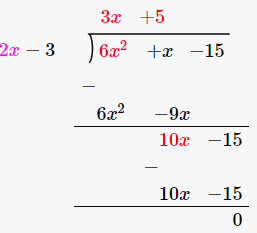Therefore, 6x2 + x – 15 = (2x – 3) (3x + 5)

4. When 3x2 – 5x + p is divided by (x – 2), the remainder is 3. Find the value of p. Also factorize the polynomial 3x2 – 5x + p – 3.

Solution:-

Let us assume x – 2 = 0

Then, x = 2

Given, f(x) = 3x2 – 5x + p

Now, substitute the value of x in f(x),

So, f(2) = 3(2)2 – 5(2) + p

= 3(4) – 10 + p

= 12 – 10 + p

= 2 + p

From the question it is given that, remainder is 3.

So, 2 + p = 3

p = 3 – 2

p = 1

Therefore, f(x) = 3x2 – 5x + 1

Consider the polynomial, 3x2 – 5x + p – 3

Now, substitute the value of p in polynomial,

= 3x2 – 5x + 1 – 3

= 3x2 – 5x – 2

Now, by factorizing the polynomial 3x2 – 5x – 2,

Dividing 3x2 – 5x – 2 by x – 2 we get,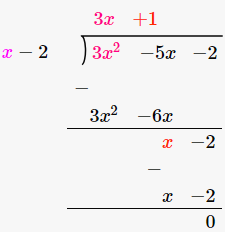Therefore, 3x2 – 5x – 2 = (x – 2) (3x + 1)

5. Prove that (5x + 4) is a factor of 5x3 + 4x2 – 5x – 4. Hence factorize the given polynomial completely.

Solution:-

Let us assume (5x + 4) = 0

Then, 5x = -4

x = -4/5

Given, f(x) = 5x3 + 4x2 – 5x – 4

Now, substitute the value of x in f(x),

So, f(-4/5) = 5(-4/5)3 + 4(-4/5)2 – 5(-4/5) – 4

= 5(-64/125) + 4 (16/25) + 4 – 4

= -64/25 + 64/25

= (-64 + 64)/25

= 0/25

= 0

Hence, (5x + 4) is a factor of 5x3 + 4x2 – 5x – 4.

So, dividing 5x3 + 4x2 – 5x – 4 by 5x + 4 we get,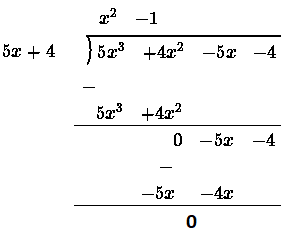Therefore, 5x3 + 4x2 – 5x – 4 = (5x + 4) (x2 – 1)

= (5x + 4) (x2 – 12)

= (5x + 4) (x + 1) (x – 1)

6. Use factor theorem to factorize the following polynomials completely:

(i) 4x3 + 4x2 – 9x – 9

Solution:-

Let us assume x = -1,

Given, f(x) = 4x3 + 4x2 – 9x – 9

Now, substitute the value of x in f(x),

f(-1) = 4(-1)3 + 4(-1)2 – 9(-1) – 9

= -4 + 4 + 9 – 9

= 0

Therefore, x + 1 is the factor of 4x3 + 4x2 – 9x – 9.

Now, dividing 4x3 + 4x2 – 9x – 9 by x + 1 we get,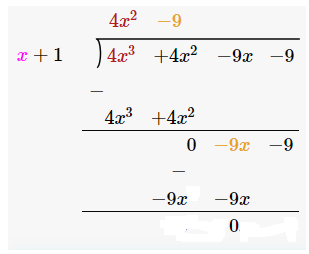Therefore, 4x3 + 4x2 – 9x – 9 = (x + 1) (4x2 – 9)

= (x + 1) ((2x)2 – (3)2)

= (x + 1) (2x + 3) (2x – 3)

(ii) x3 – 19x – 30

Solution:-

Let us assume x = -2,

Given, f(x) = x3 – 19x – 30

Now, substitute the value of x in f(x),

f(-1) = (-2)3 – 19(-2) – 30

= -8 + 38 – 30

= -38 + 38

= 0

Therefore, x + 2 is the factor of x3 – 19x – 30.

Now, dividing x3 – 19x – 30 by x + 2 we get,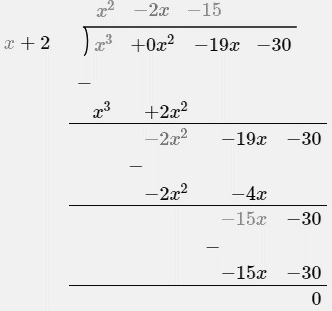Therefore, x3 – 19x – 30 = (x + 2)(x2 – 2x – 15)

= (x + 2) (x2 – 5x + 3x – 15)

= (x + 2) (x – 5) (x + 3)

7. If x3 – 2x2 + px + q has a factor (x + 2) and leaves a remainder 9, when divided by (x + 1), find the values of p and q. With these values of p and q, factorize the given polynomial completely.

Solution:-

From the question it is given that, (x + 2) is a factor of the expression x3 – 2x2 + px + q

Then, f(x) = x3 – 2x2 + px + q

Let assume x + 2 = 0

Then, x = -2

Now, substitute the value of x in f(x),

f(-2) = (-2)3 – 2(-2)2 + p(-2) + q

= -8 – 8 – 2p + q

= -16 – 2p + q

2p – q = – 16 … [equation (i)]

Now, consider (x + 1)

Then, f(x) = x3 – 2x2 + px + q

Let assume x + 1 = 0

Then, x = -1

Now, substitute the value of x in f(x),

f(-1) = (-1)3 – 2(-1)2 + p(-1) + q

= -1 – 2 –p + q

= – 3 – p + q

Given, remainder is 9

So, -3 – p + q = 9

– p + q = 9 + 3

-p + q = 12 … [equation (ii)]

Now, adding equation (i) and equation (ii) we get,

(2p – q) + (-p + q) = – 16 + 12

2p – q – p + q = -4

P = -4

Consider the equation (ii) to find out ‘b’.

– p + q = 12

-(-4) + q = 12

4 + q = 12

q = 12 – 4

q = 8

Therefore, by substituting the value of p and q f(x) = x3 – 2x2 – 4x + 8

Dividing f(x) be (x + 2) we get,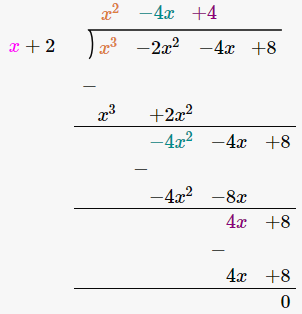x3 – 2x2 – 4x + 8 = (x + 2) (x2 – 4x + 4)

= (x + 2) (x2 – 2 × x (-2) + 22)

= (x + 2) (x – 2)2

8. If (x + 3) and (x – 4) are factors of x3 + ax2 – bx + 24, find the values of a and b: With these values of a and b, factorize the given expression.

Solution:-

Let us assume x + 3 = 0

Then, x = -3

Given, f(x) = x3 + ax2 – bx + 24

Now, substitute the value of x in f(x),

f(-3) = (-3)3 + a(-3)2 – b(-3) + 24

= -27 + 9a + 3b + 24

= 9a + 3b – 3

Dividing all terms by 3 we get,

= 3a + b – 1

From the question, (x + 3) is a factor of x3 + ax2 – bx + 24.

Therefore, remainder is 0.

f(x) = 0

3a + b – 1 = 0

3a + b = 1 … [equation (i)]

Now, assume x – 4 = 0

Then, x = 4

Given, f(x) = x3 + ax2 – bx + 24

Now, substitute the value of x in f(x),

f(4) = 43 + a(4)2 – b(4) + 24

= 64 + 16a – 4b + 24

= 88 + 16a – 4b

Dividing all terms by 4 we get,

= 22 + 4a – b

From the question, (x – 4) is a factor of x3 + ax2 – bx + 24.

Therefore, remainder is 0.

f(x) = 0

22 + 4a – b = 0

4a – b = – 22 … [equation (ii)]

Now, adding both equation (i) and equation (ii) we get,

(3a + b) + (4a – b) = 1 – 22

3a + b + 4a – b = – 21

7a = – 21

a = -21/7

a = -3

Consider the equation (i) to find out ‘b’.

3a + b = 1

3(-3) + b = 1

-9 + b = 1

b = 1 + 9

b = 10

Therefore, value of a = -3 and b = 10.

Then, by substituting the value of a and b f(x) = x3 – 3x2 – 10x + 24

(x + 3) (x – 4)

= x(x – 4) + 3(x – 4)

= x2 – 4x + 3x – 12

= x2 – x – 12

Dividing f(x) by x2 – x – 12 we get,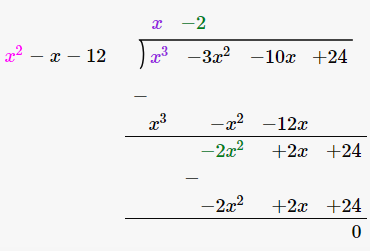Therefore, x3 – 3x2 – 10x + 24 = (x2 – x – 12) (x – 2)

= (x + 3) (x – 4) (x – 2)

9. If 2x3 + ax2 – 11x + b leaves remainder 0 and 42 when divided by (x – 2) and (x – 3) respectively, find the values of a and b. With these values of a and b, factorize the given expression.

Solution:-

Let us take x – 2 = 0

Then, x = 2

Given, f(x) = 2x3 + ax2 – 11x + b

Now, substitute the value of x in f(x),

f(2) = 2(2)3 + a(2)2 – 11(2) + b

= 16 + 4a – 22 + b

= – 6 + 4a + b

Given, remainder is 0.

So, -6 + 4a + b = 0

4a + b = 6 … [equation (i)]

Now, consider (x – 3)

Assume x – 3 = 0

Then, x = 3

Given, f(x) = 2x3 + ax2 – 11x + b

Now, substitute the value of x in f(x),

f(2) = 2(3)3 + a(3)2 – 11(3) + b

= 54 + 9a – 33 + b

= 21 + 9a + b

Given, remainder is 42.

So, 21 + 9a + b = 42

9a + b = 42 – 21

9a + b = 21 … [equation (ii)]

Now, subtracting equation (i) from equation (ii) we get,

(9a + b) – (4a + b) = 21 – 6

9a + b – 4a – b = 15

5a = 15

a = 15/5

a = 3

Consider the equation (i) to find out ‘b’.

4a + b = 6

4(3) + b = 6

12 + b = 6

b = 6 – 12

b = -6

Then, by substituting the value of a and b f(x) = 2x3 + 3x2 – 11x – 6

Given that remainder is 0 for, (x – 2) is a factor of f(x).

So, dividing f(x) by (x – 2)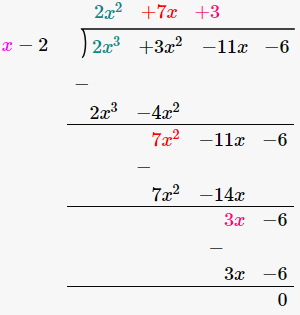Therefore, 2x3 + 3x2 – 11x – 6 = (x – 2) (2x2 + 7x + 3)

= (x – 2) (2x2 + 6x + x + 3)

= (x – 2) (2x2 + 6x + x + 3)

= (x – 2) (2x (x + 3) + 1(x + 3))

= (x – 2) (x + 3) (2x + 1)

10. If (2x + 1) is a factor of both the expressions 2x2 – 5x + p and 2x2 + 5x + q, find the value of p and q. Hence find the other factors of both the polynomials.

Solution:-

Let us assume 2x + 1 = 0

Then, 2x = -1

x = -½

Given, p(x) = 2x2 – 5x + p

Now, substitute the value of x in p(x),

p (-½) = 2 (-½)2 – 5(-½) + p

= 2(1/4) + 5/2 + p

= ½ + 5/2 + p

= 6/2 + p

= 3 + p

From the question it is given that, (2x + 1) is a factor of both the expressions 2x2 – 5x + p

So, remainder is 0.

Then, 3 + p = 0

p = – 3

Now consider q(x) = 2x2 + 5x + q

Substitute the value of x in q(x)

q (-½) = 2 (-½)2 + 5(-½) + q

= 2(1/4) – 5/2 + q

= ½ – 5/2 + q

= (1 – 5)/2 + q

= -4/2 + q

= q – 2

From the question it is given that, (2x + 1) is a factor of both the expressions 2x2 + 5x + q

So, remainder is 0.

q – 2 = 0

q = 2

Therefore, p = – 3 and q = 2

P(x) = 2x2 – 5x – 3

q(x) = 2x2 + 5x + 2

Then, divide p(x) by 2x + 1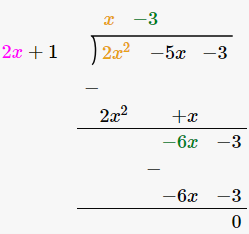Therefore, 2x2 – 5x – 3 = (2x + 1) (x – 3)

Now, divide q(x) by 2x + 1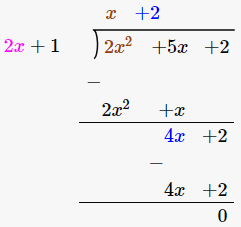Therefore, 2x2 + 5x + 2 = (2x + 1) (x + 2)

11. If a polynomial f(x)= X4-2x3 + 3x2– ax + b leaves reminder 5 and 19 when divided by (x-1) and (x+1) respectively, Find the values of a and b. Hence determined the reminder when f(x) is divided by (x-2).

Solution:-

From the question it is given that,

f(x) = x4 – 2x3 +3x2 – ax + b

Factor (x – 1) leaves remainder 5,

Factor (x + 1) leaves remainder 19,

Where x = 1 and x = – 1

f(-1) = (-1)4 – 2(-1)3 + 3(-1)2 – a(-1) + b = 19

1 – 2(-1) + 3(1) – a(-1) + b = 19

1 + 2 + 3 + a + b = 19

6 + a + b = 19

a + b = 19 – 6

a + b = 13 … [equation (i)]

f(1) = (1)4 – 2(1)3 + 3(1)2 – a(1) + b = 5

1 – 2(1) + 3(1) – a(1) + b = 5

1 – 2 + 3 – a + b = 5

2 – a + b = 5

– a + b = 5 – 2

– a + b = 3 … [equation (ii)]

Now, subtracting equation (ii) from equation (i) we get,

(a + b) – (- a + b) = 13 – 3

a + b + a – b = 10

2a = 10

a = 10/2

a = 5

To find out the value of b, substitute the value of a in equation (i) we get,

a + b = 13

5 + b = 13

b = 13 – 5

b = 8

Therefore, value of a = 5 and b = 8

12. When a polynomial f(x) is divided by (x – 1), the remainder is 5 and when it is, divided by (x – 2), the remainder is 7. Find the remainder when it is divided by (x – 1) (x – 2).

Solution:-

From the question it is given that,

Polynomial f(x) is divided by (x – 1),

Remainder = 5

Let us assume x – 1 = 0

x = 1

f(1) = 5

and the divided be (x – 2), remainder = 7

let us assume x – 2 = 0

x = 2

Therefore, f(2) = 7

So, f(x) = (x – 1) (x – 2) q(x) + ax + b

Where, q(x) is the quotient and ax + b is remainder,

Now put x = 1, we get,

f(1) = (1 – 1)(1 – 2)q(1) + (a × 1) + b

a + b = 5 … [equation (i)]

x = 2,

f(2) = (2 – 1)(2 – 2)q(2) + (a × 2) + b

2a + b = 7 … [equation (ii)]

Now subtracting equation (i) from equation (ii) we get,

(2a + b) – (a + b) = 7 – 5

2a + b – a – b = 2

a = 2

To find out the value of b, substitute the value of a in equation (i) we get,

a + b = 5

2 + b = 5

b = 5 – 2

b = 3

Therefore, the remainder = ax + b

Then, 2x + 3